# RS Aggarwal Solutions for Class 8 Maths Chapter 6 - Operations on Algebraic Expressions

RS Aggarwal Solutions For Class 8 Maths Chapter 6 Operations on Algebraic Expressions are provided here. You can download the pdf of RS Aggarwal Solutions for Class 8 Maths Chapter 6 Operations on Algebraic Expression from the given links. Class 8 is an important phase of a student’s life. It is crucial concepts that are taught in Class 8 are understood thoroughly as these concepts are continued in Class 9 and 10 and are also the foundation for higher studies. Here we will learn about the basic operations that are addition, subtraction, multiplication and division on algebraic expressions.

RS Aggarwal Solutions helps students to get a good score in the examinations, while also providing extensive knowledge about the subject, as Class 8 is a critical stage in their academic career. Therefore, we at BYJU’S provide answers to all questions uniquely and briefly.

## Download pdf of RS Aggarwal Solutions For Class 8 Maths Chapter 6 Operations on Algebraic Expressions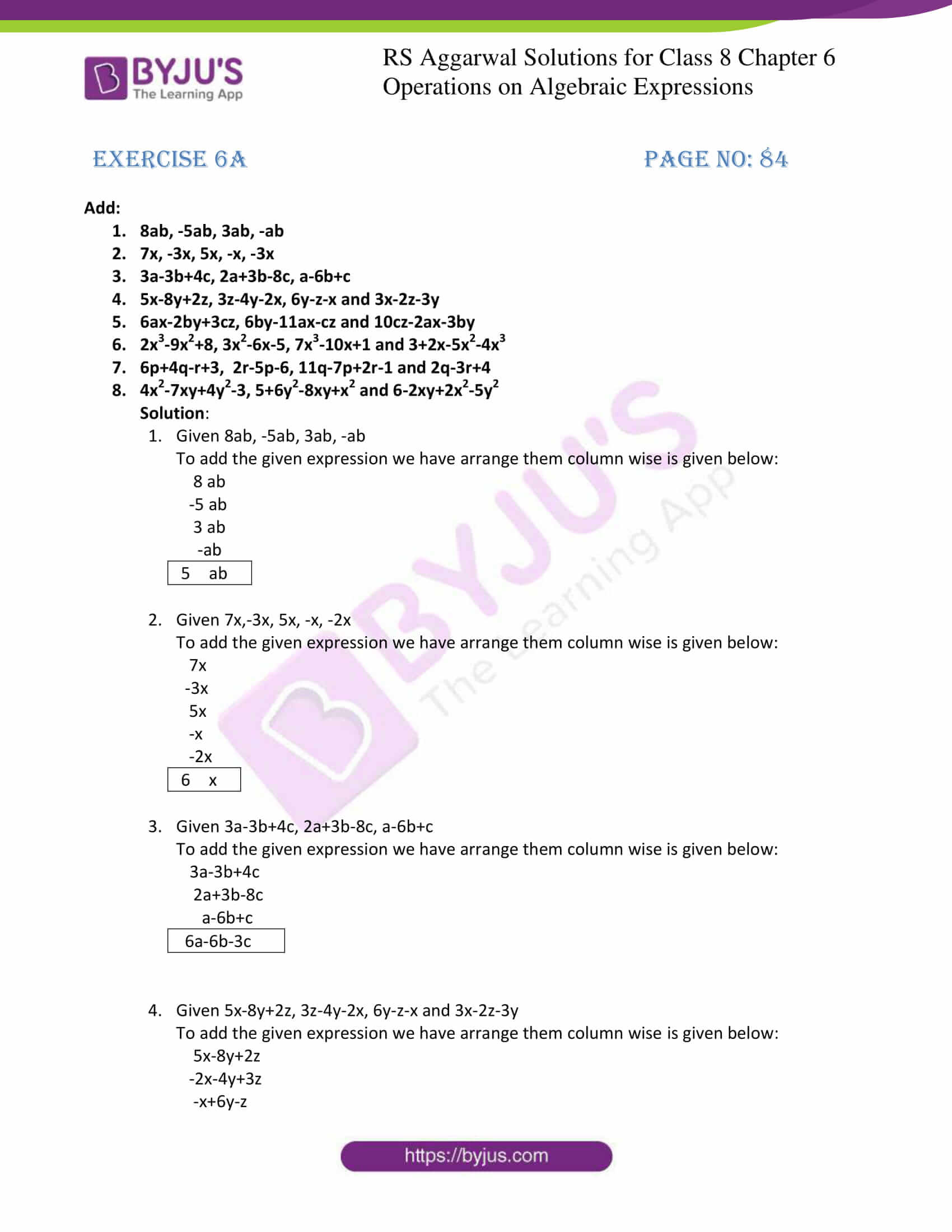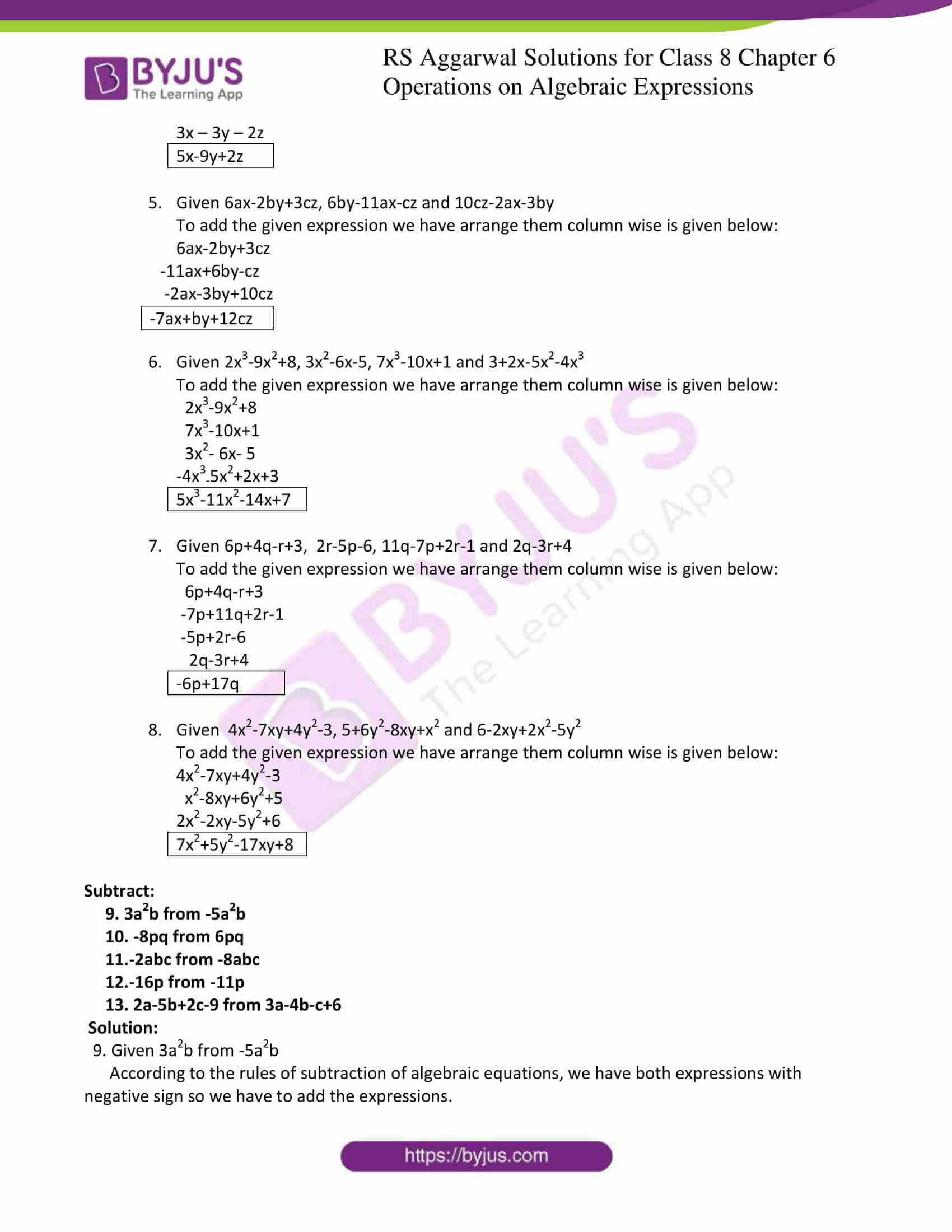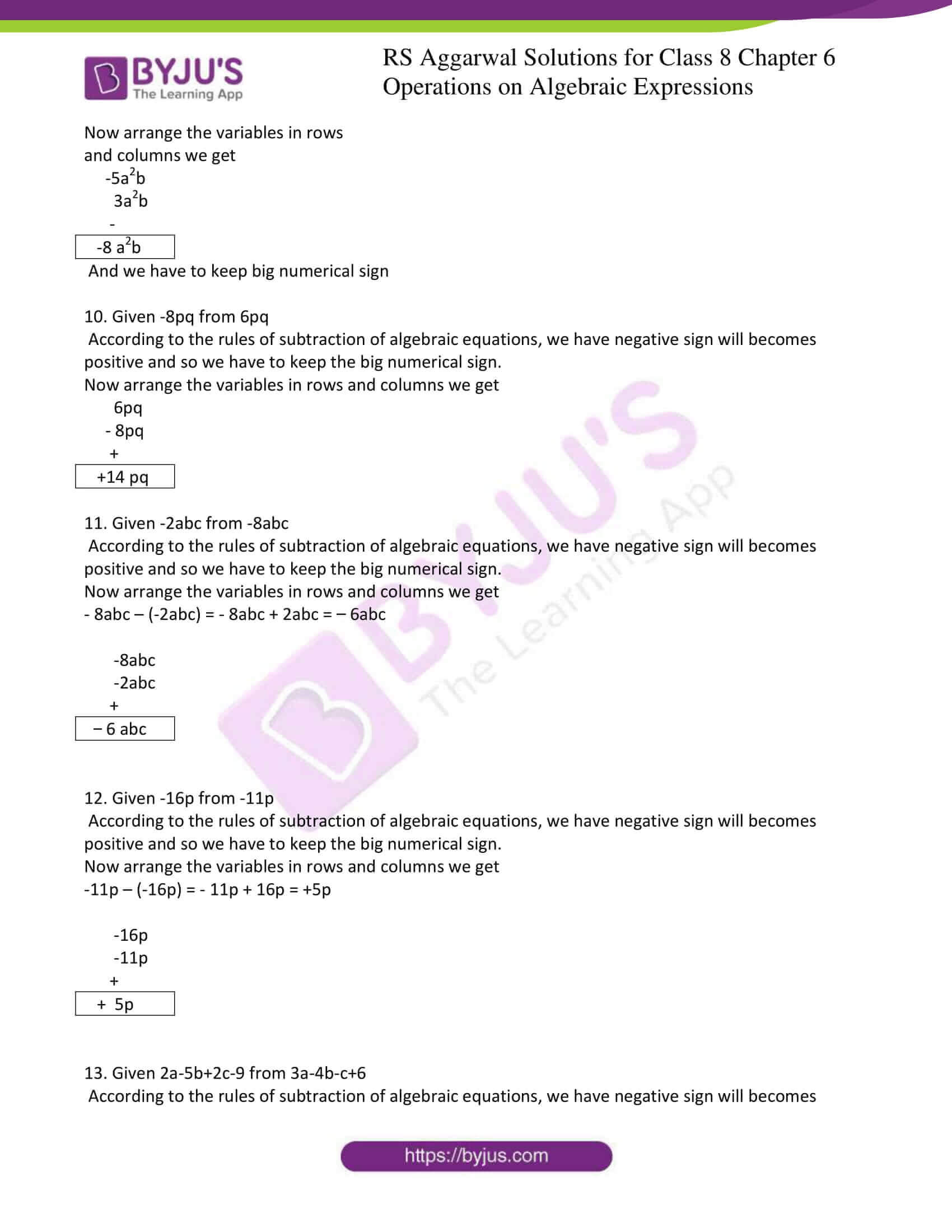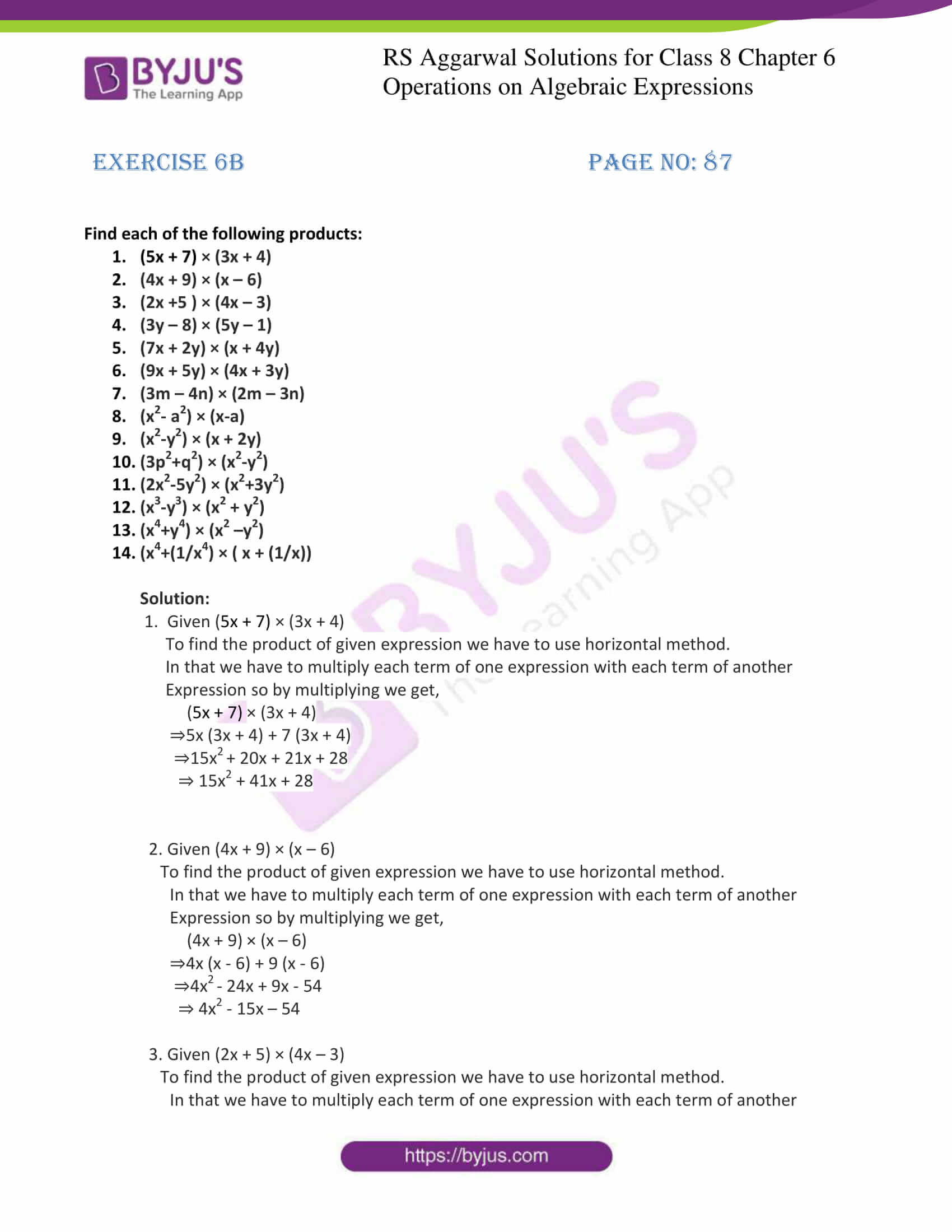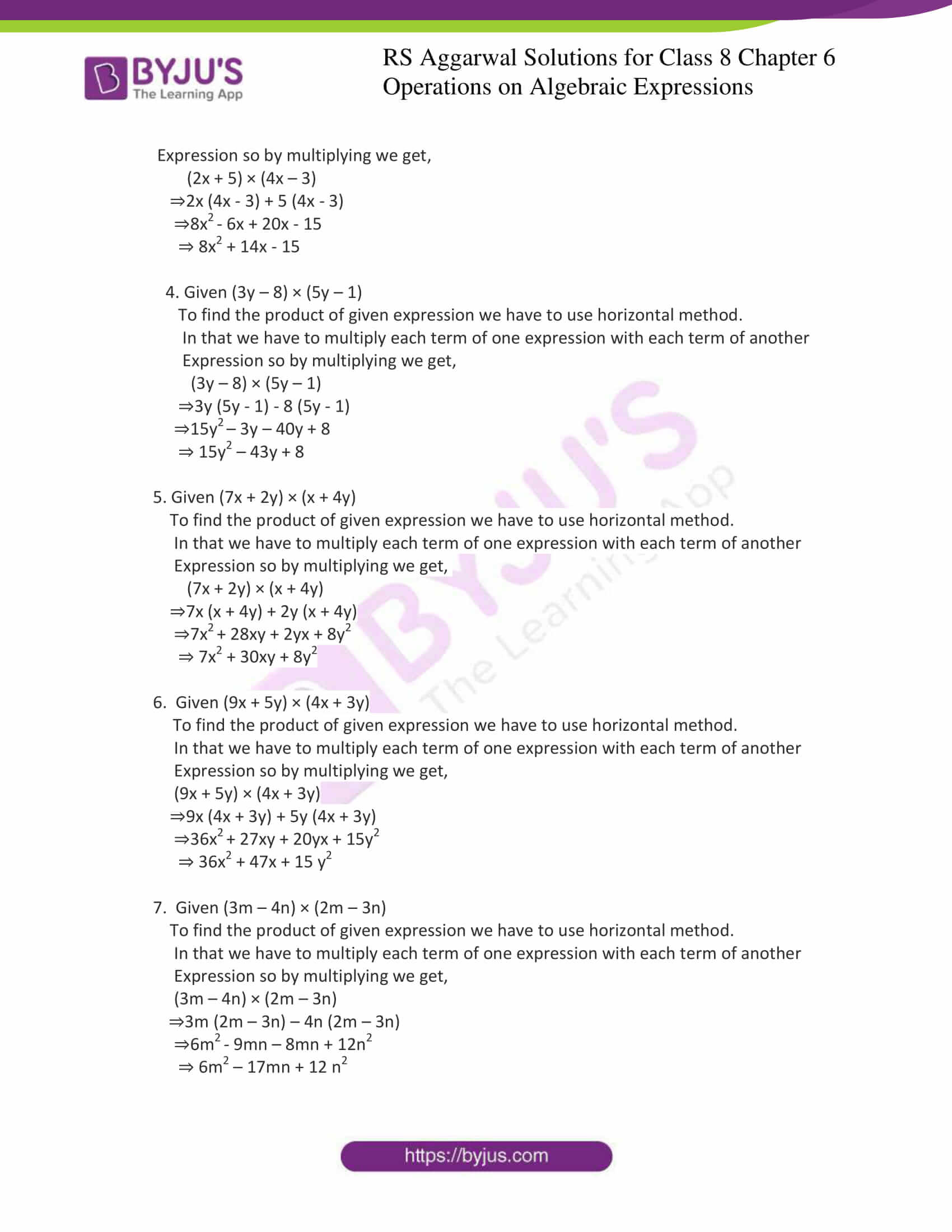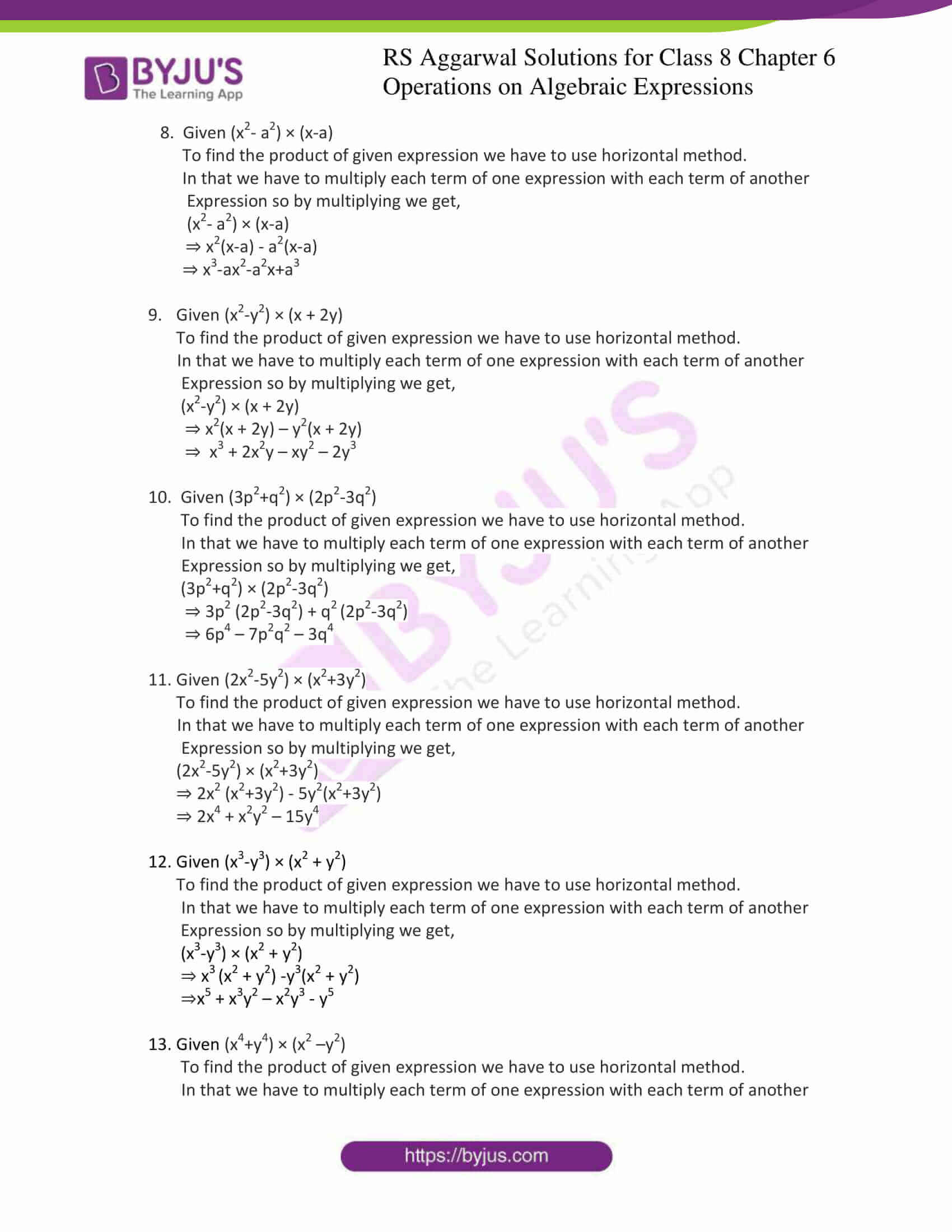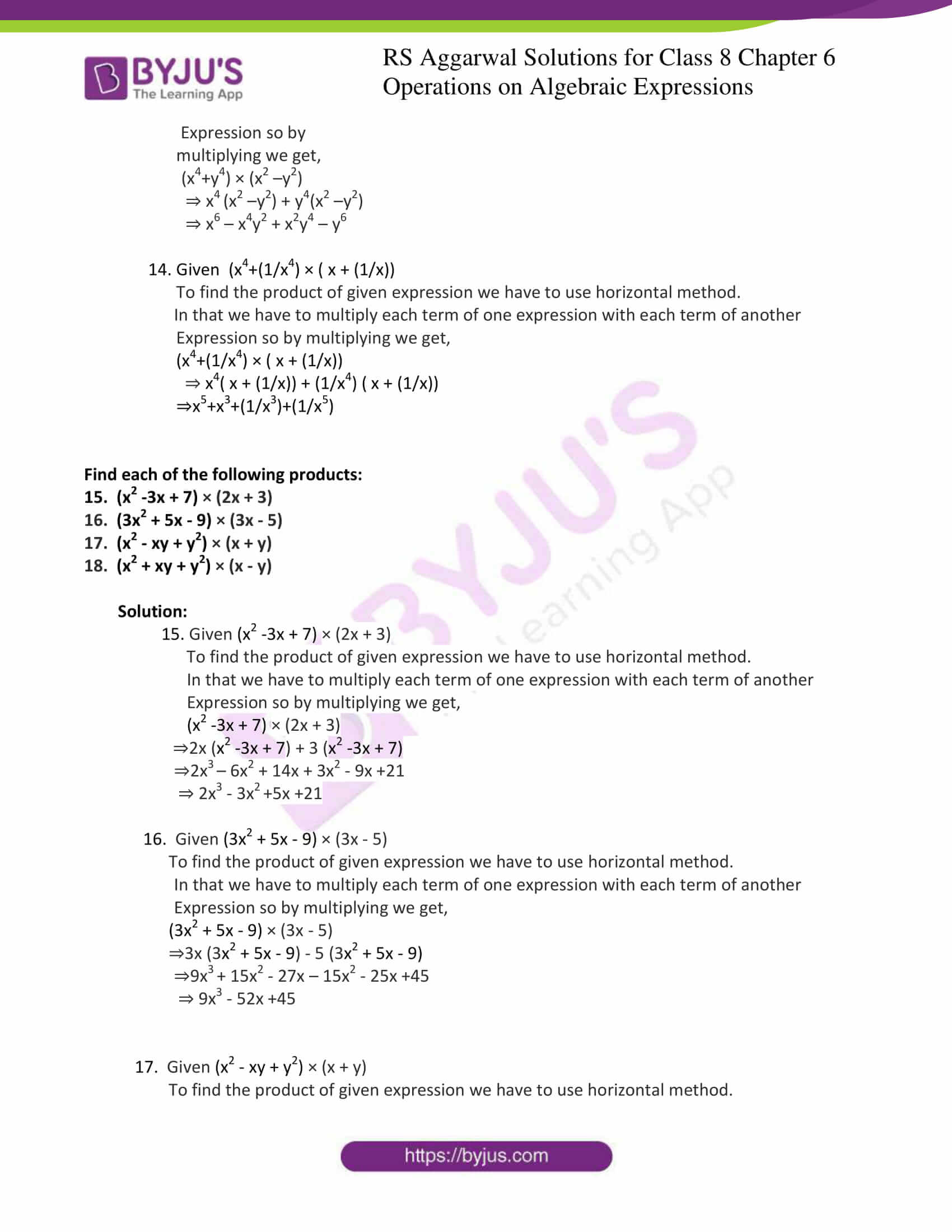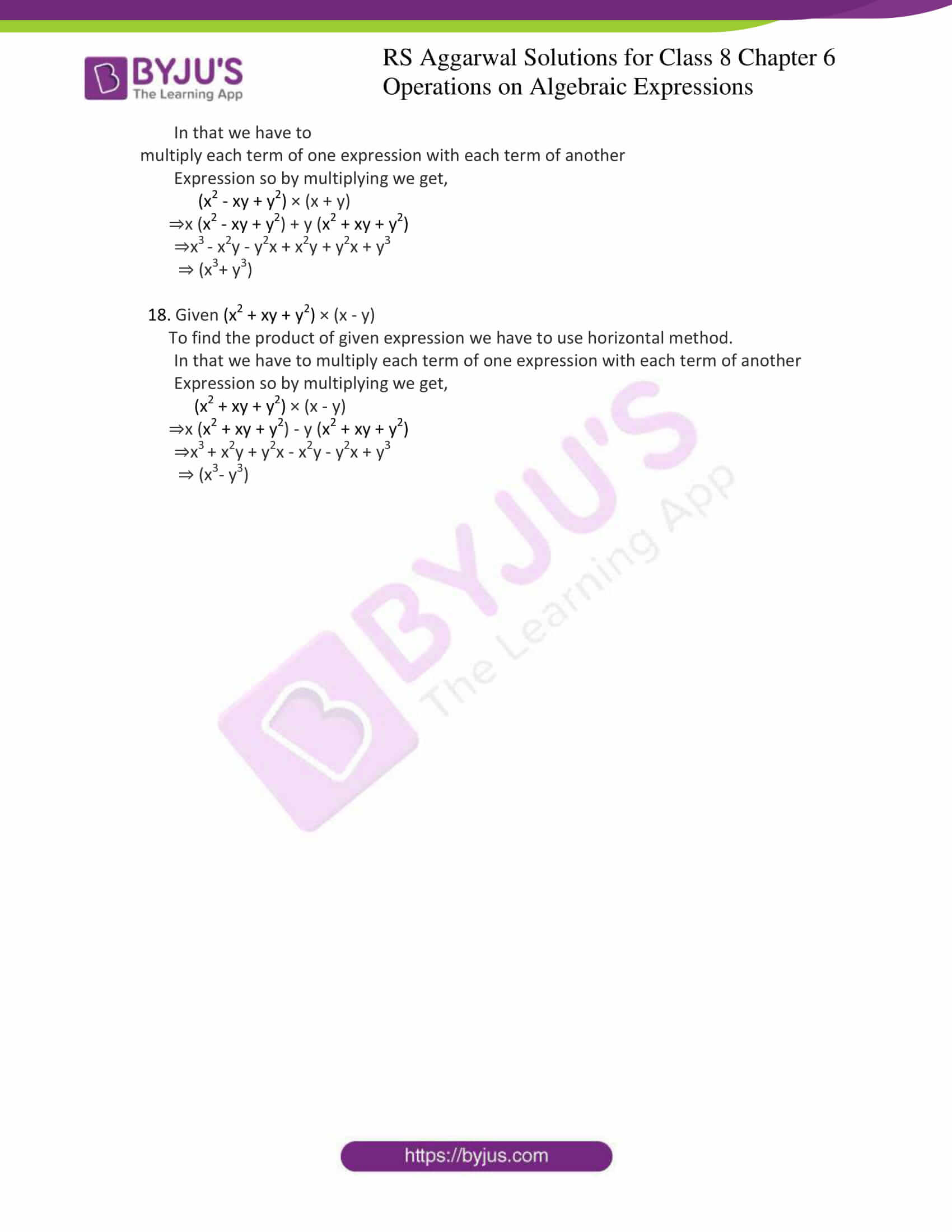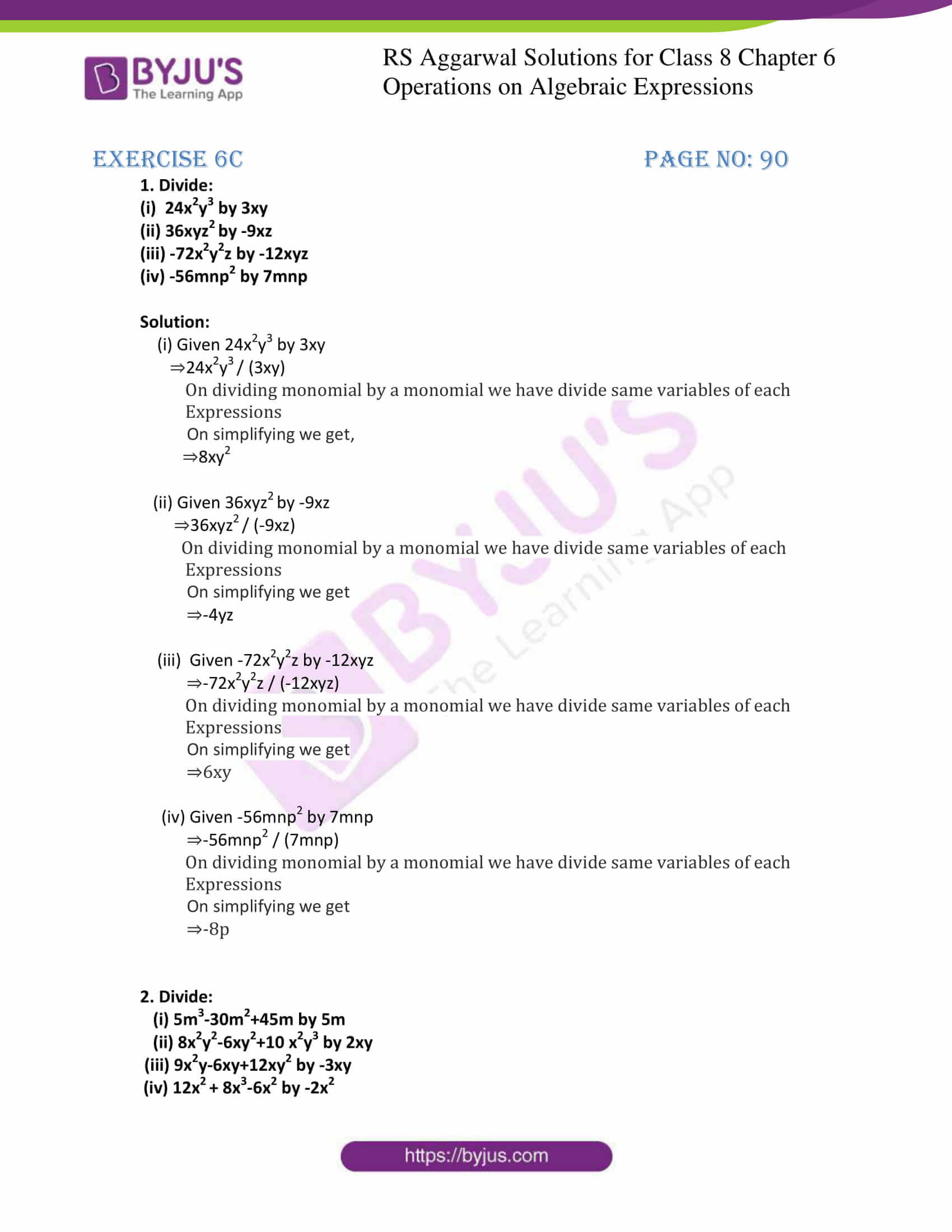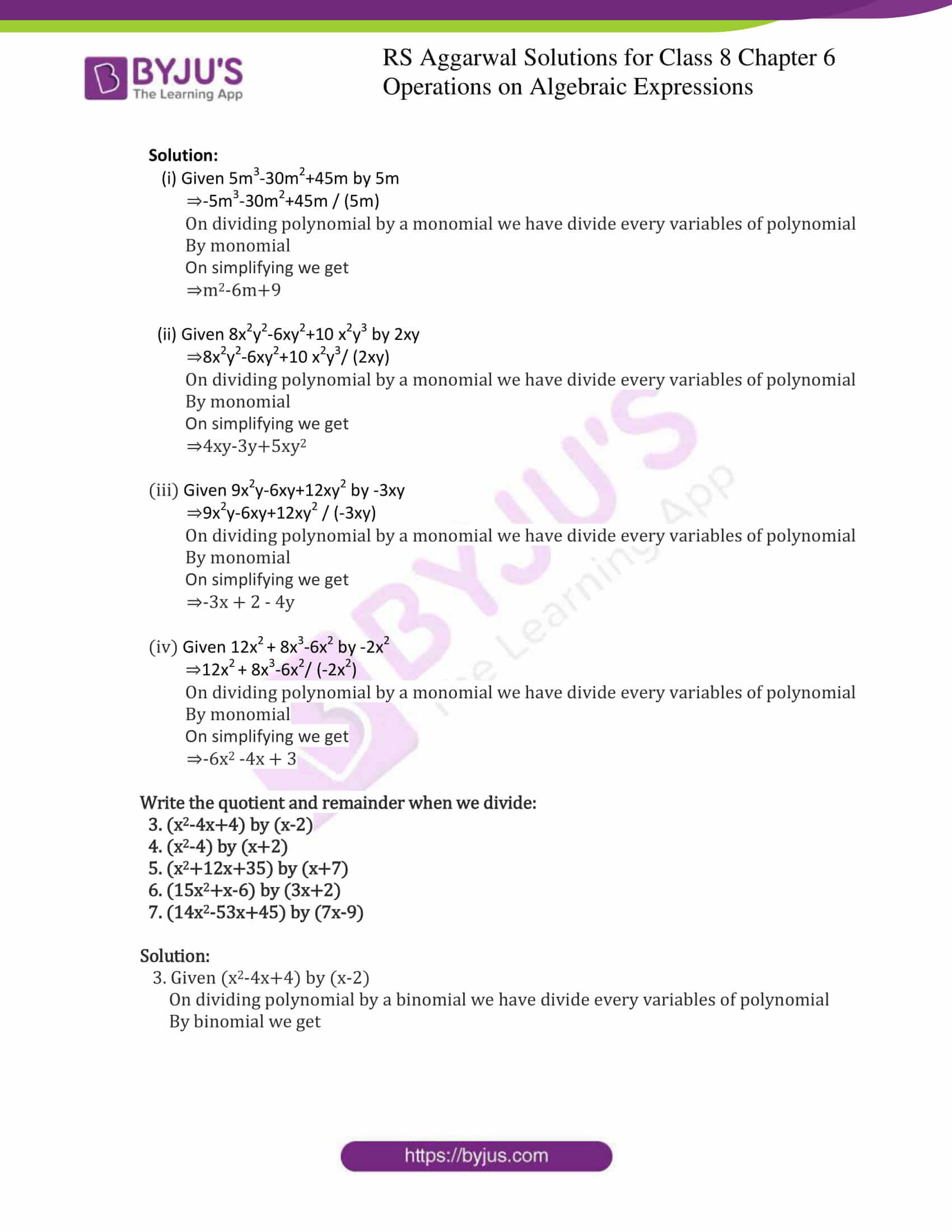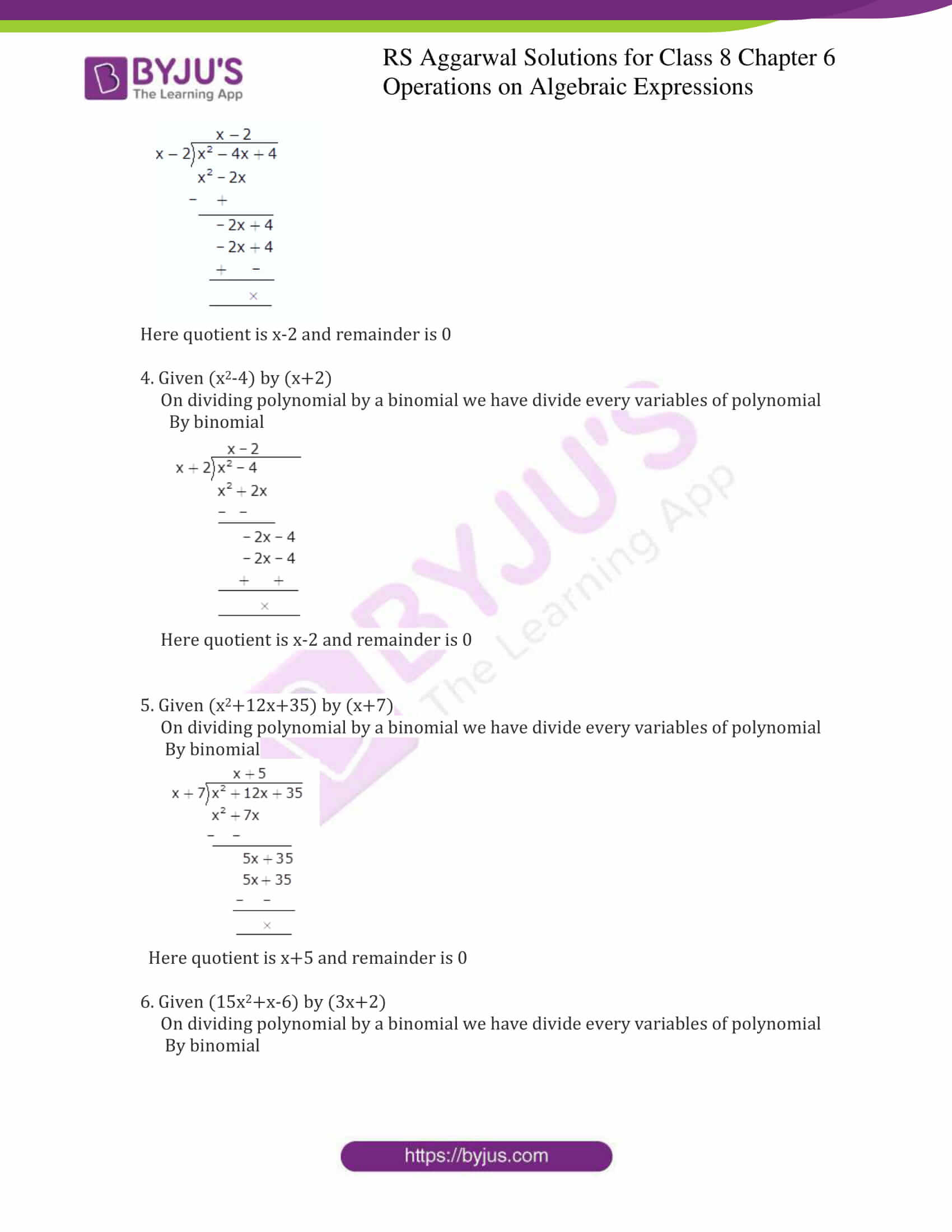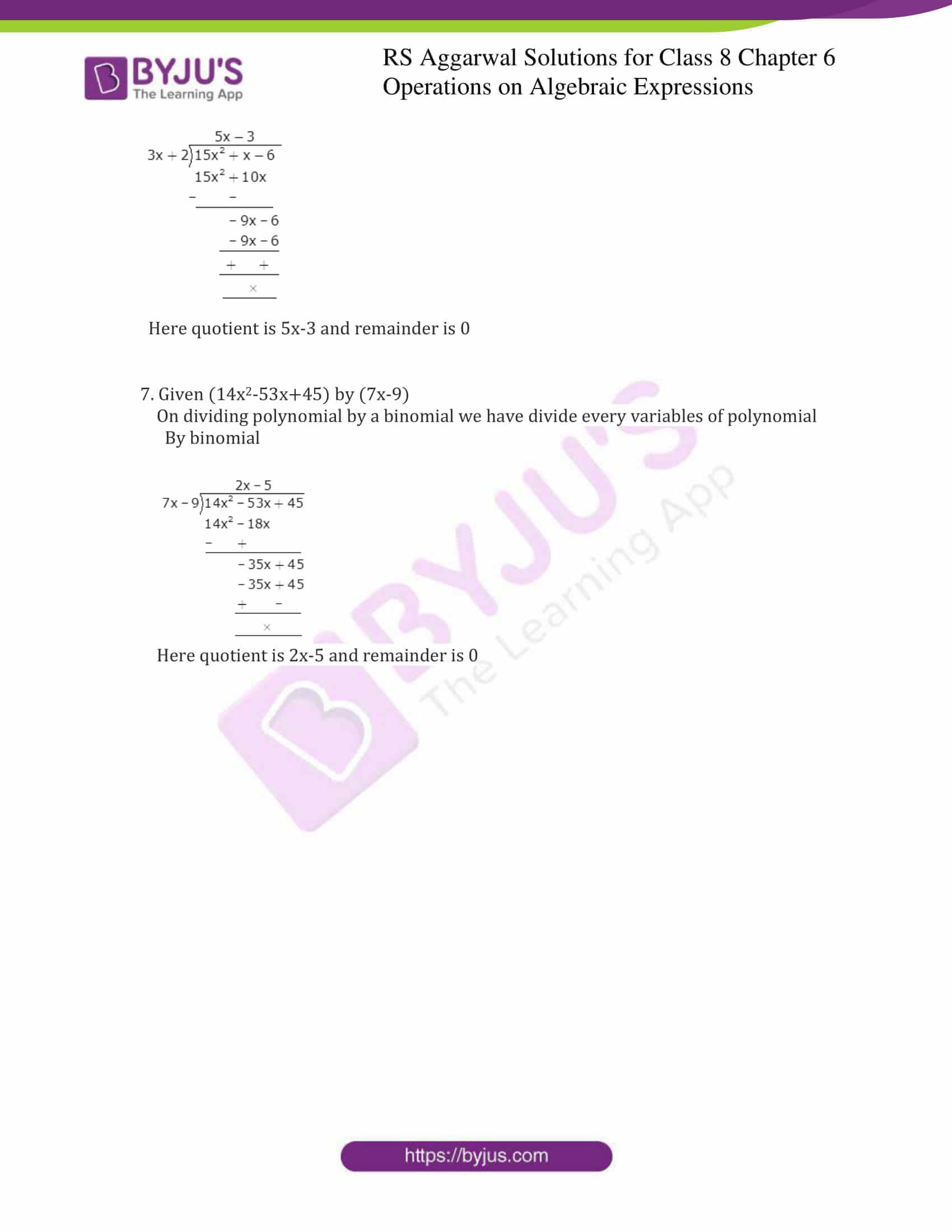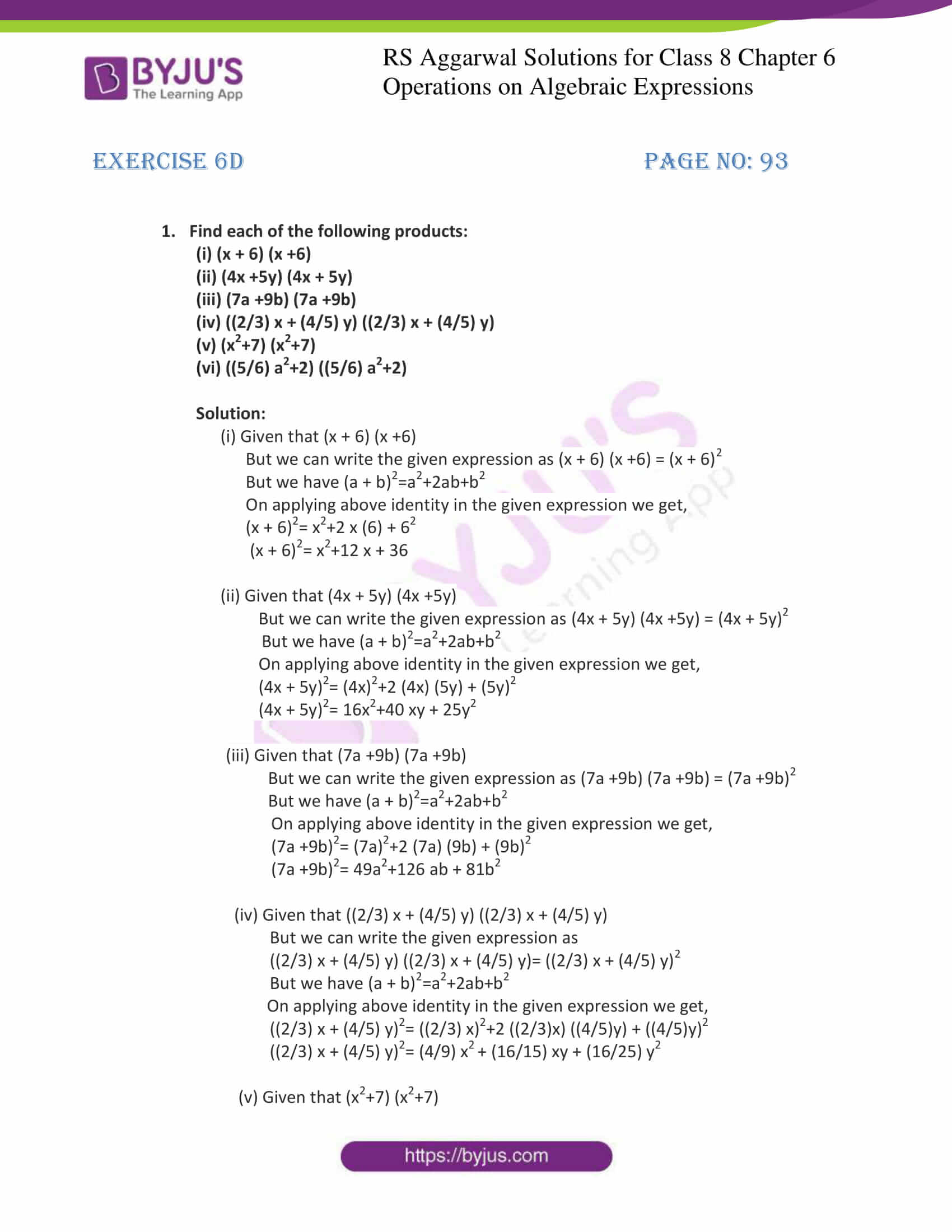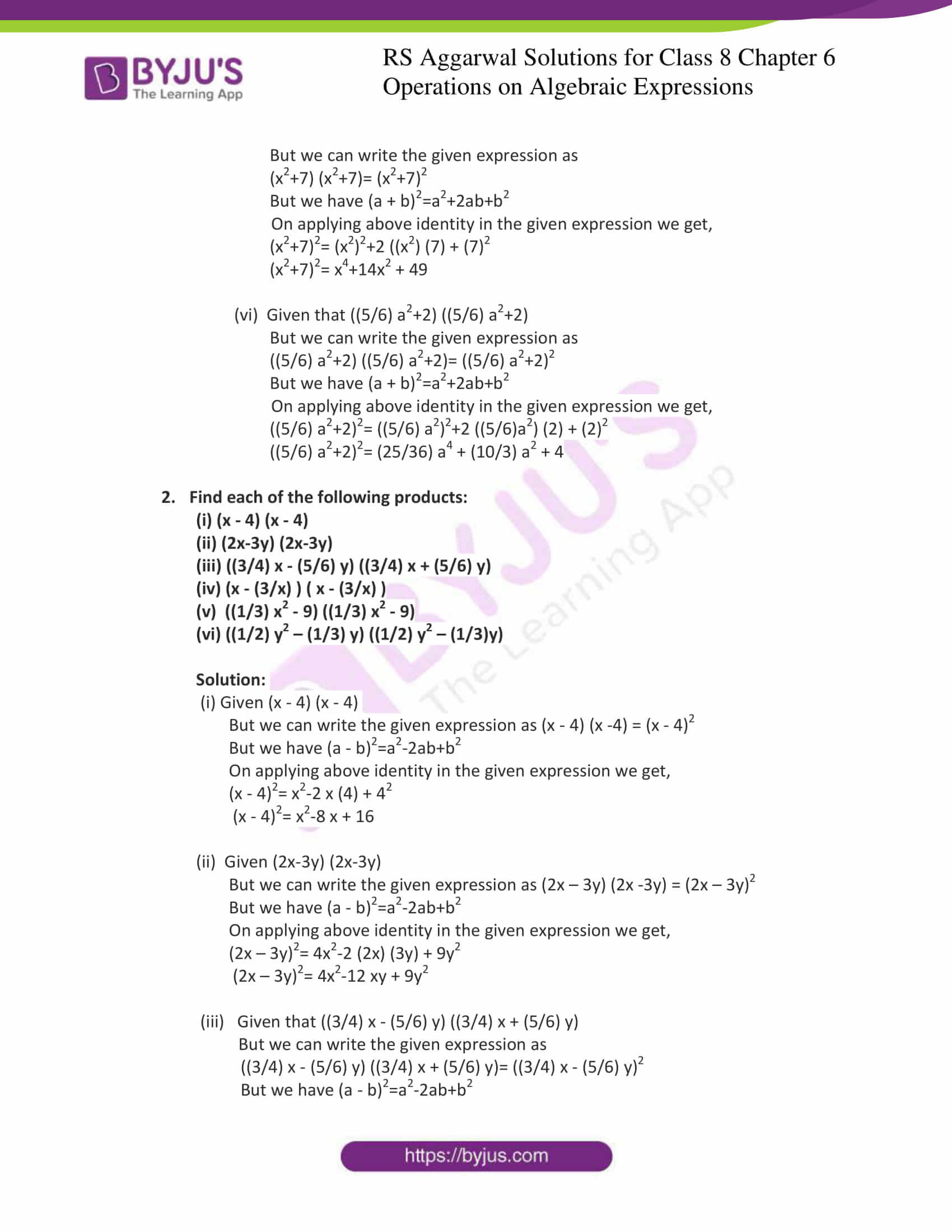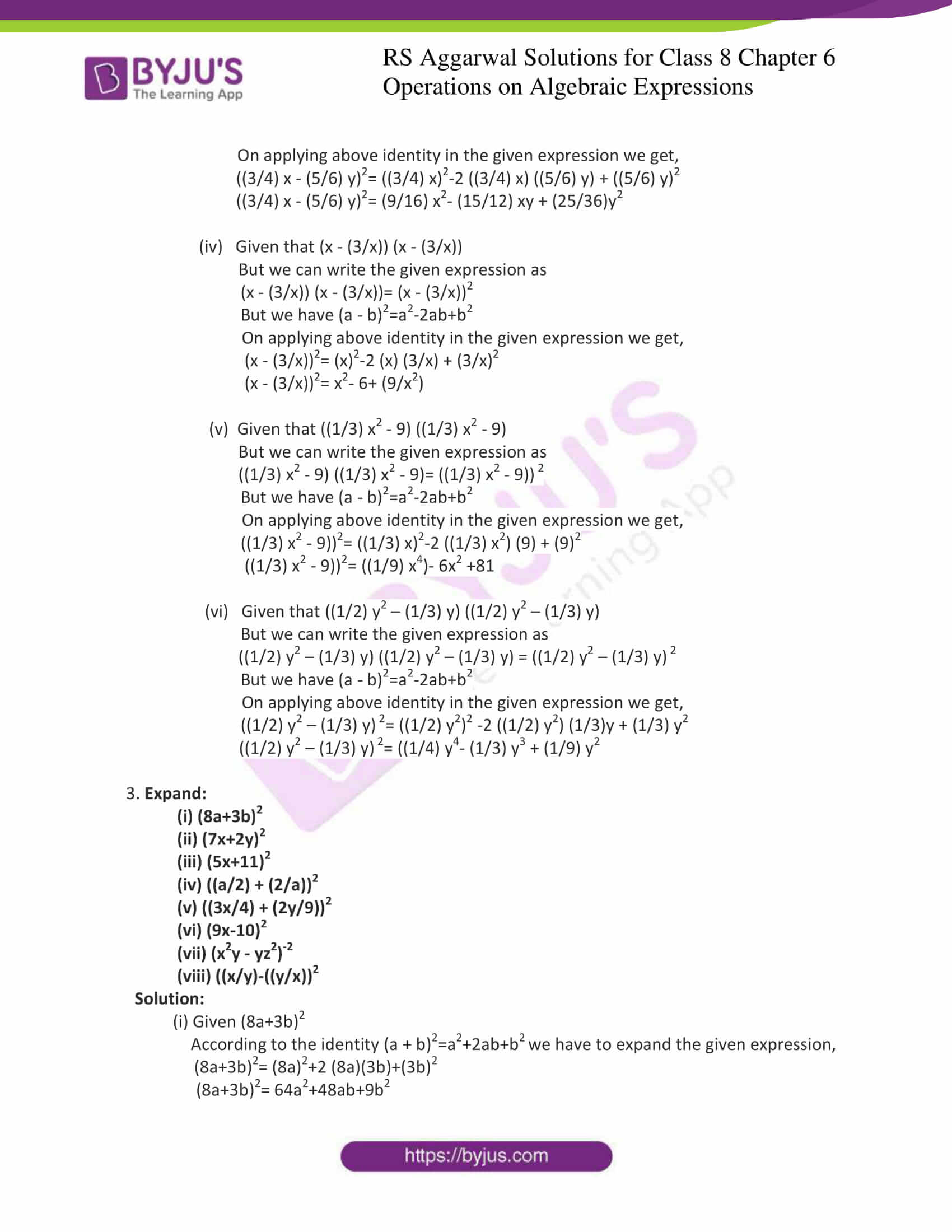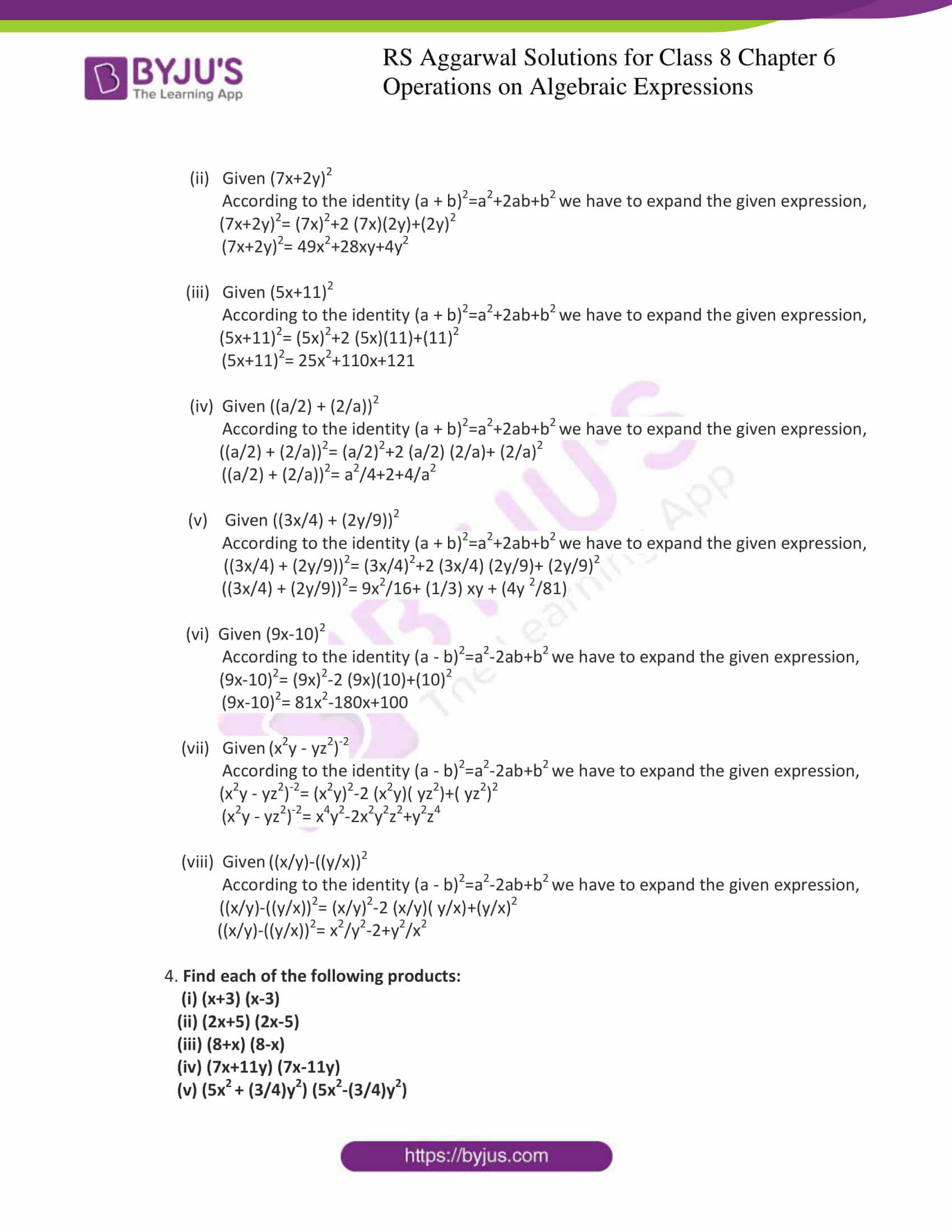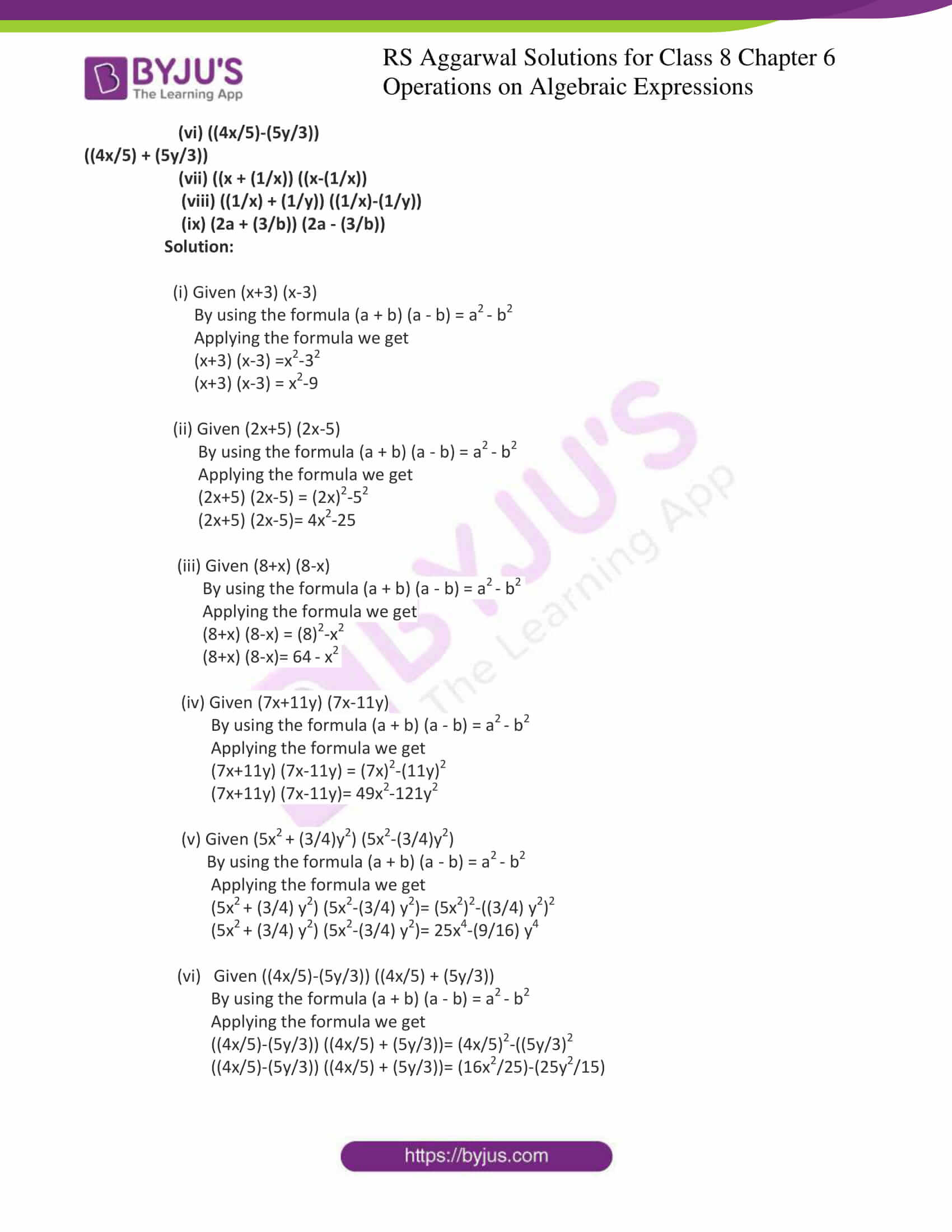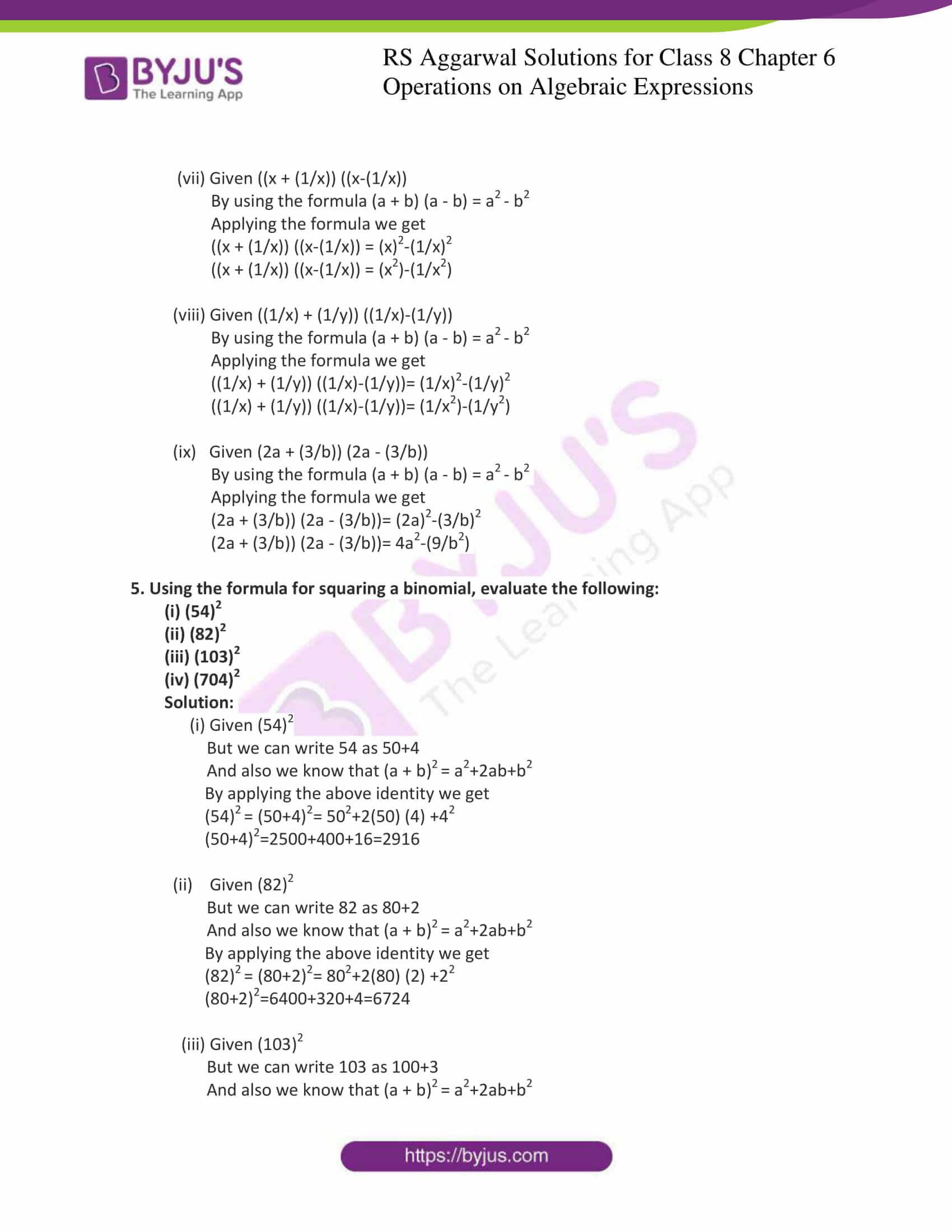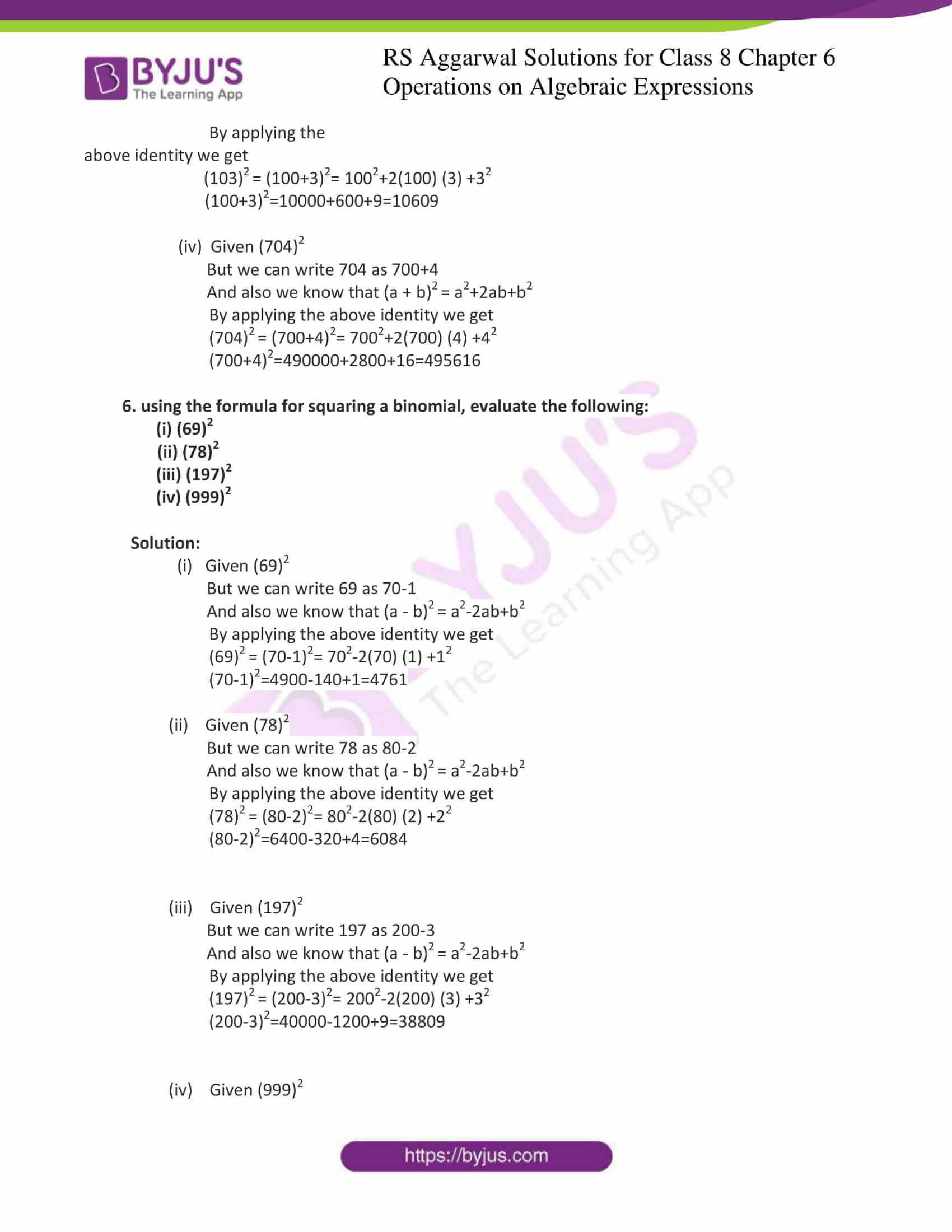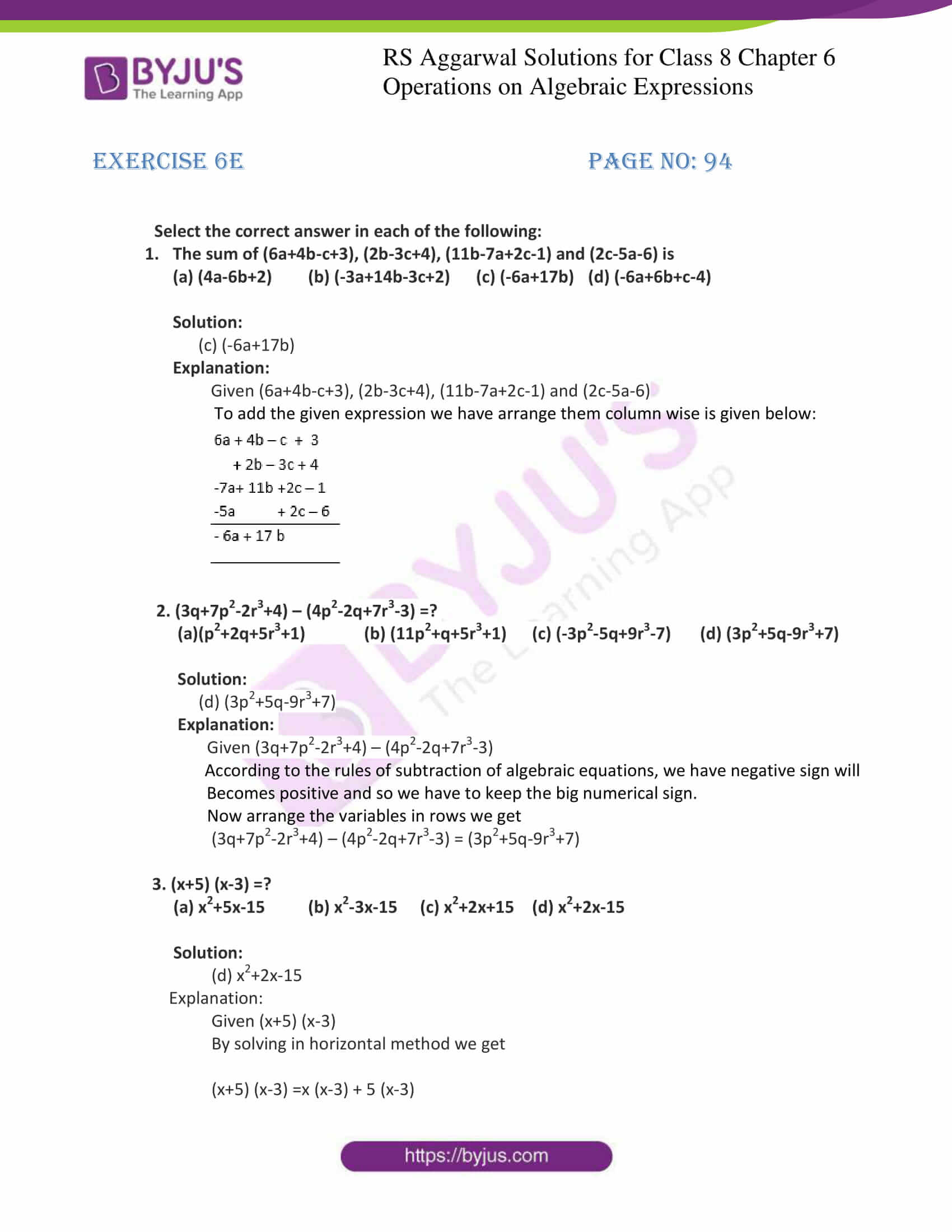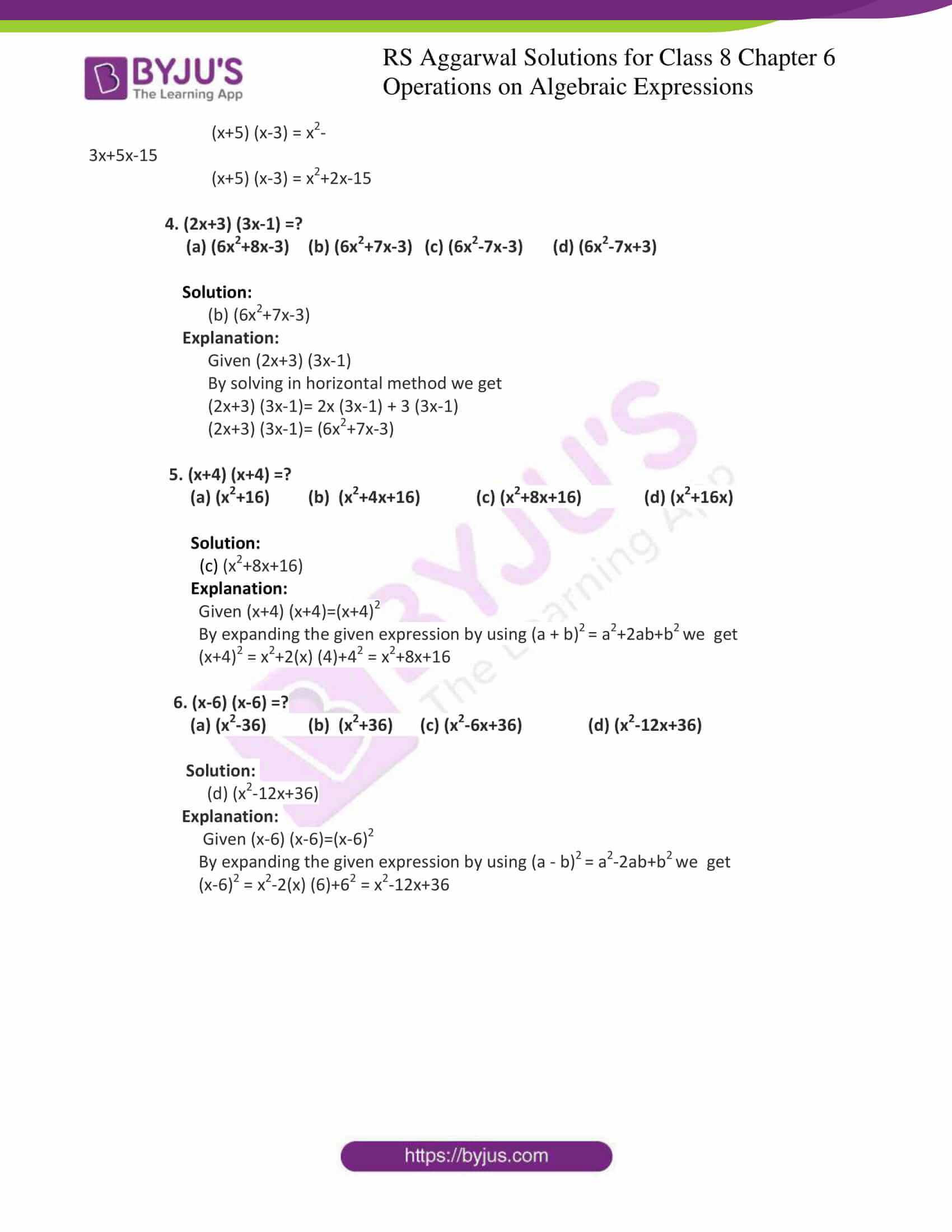## Exercise 6A

1. 8ab, -5ab, 3ab, -ab

2. 7x, -3x, 5x, -x, -3x

3. 3a-3b+4c, 2a+3b-8c, a-6b+c

4. 5x-8y+2z, 3z-4y-2x, 6y-z-x and 3x-2z-3y

5. 6ax-2by+3cz, 6by-11ax-cz and 10cz-2ax-3by

6. 2x3-9x2+8, 3x2-6x-5, 7x3-10x+1 and 3+2x-5x2-4x3

7. 6p+4q-r+3, 2r-5p-6, 11q-7p+2r-1 and 2q-3r+4

8. 4x2-7xy+4y2-3, 5+6y2-8xy+x2 and 6-2xy+2x2-5y2

Solution:

1. Given 8ab, -5ab, 3ab, -ab

To add the given expression we have arrange them column wise is given below:

8 ab

-5 ab

3 ab

-ab

 5ab

2. Given 7x,-3x, 5x, -x, -2x

To add the given expression we have arrange them column wise is given below:

7x

-3x

5x

-x

-2x

 6x

3. Given 3a-3b+4c, 2a+3b-8c, a-6b+c

To add the given expression we have arrange them column wise is given below:

3a-3b+4c

2a+3b-8c

a-6b+c

 6a-6b-3c

4. Given 5x-8y+2z, 3z-4y-2x, 6y-z-x and 3x-2z-3y

To add the given expression we have arrange them column wise is given below:

5x-8y+2z

-2x-4y+3z

-x+6y-z

3x – 2z – 3y

 5x-9y+2z

5. Given 6ax-2by+3cz, 6by-11ax-cz and 10cz-2ax-3by

To add the given expression we have arrange them column wise is given below:

6ax-2by+3cz

-11ax+6by-cz

-2ax-3by+10cz

 -7ax+by+12cz

6. Given 2x3-9x2+8, 3x2-6x-5, 7x3-10x+1 and 3+2x-5x2-4x3

To add the given expression we have arrange them column wise is given below:

2x3-9x2+8

7x3-10x+1

3x2– 6x- 5

-4x3-5x2+2x+3

 5x3-11x2-14x+7

7. Given 6p+4q-r+3, 2r-5p-6, 11q-7p+2r-1 and 2q-3r+4

To add the given expression we have arrange them column wise is given below:

6p+4q-r+3

-7p+11q+2r-1

-5p+2r-6

2q-3r+4

 -6p+17q

8. Given 4x2-7xy+4y2-3, 5+6y2-8xy+x2 and 6-2xy+2x2-5y2

To add the given expression we have arrange them column wise is given below:

4x2-7xy+4y2-3

x2-8xy+6y2+5

2x2-2xy-5y2+6

 7x2+5y2-17xy+8

Subtract:

9. 3ax2b from -5ax2b

10. -8pq from 6pq

11.-2abc from -8abc

12.-16p from -11p

13. 2a-5b+2c-9 from 3a-4b-c+6

Solution:

9. Given 3ax2b from -5ax2b

According to the rules of subtraction of algebraic equations, we have both expressions with negative sign so we have to add the expressions.

Now arrange the variables in rows and columns we get

-5ax2b

3ax2b

 -8 ax2b

And we have to keep big numerical sign

10. Given -8pq from 6pq

According to the rules of subtraction of algebraic equations, we have negative sign will becomes positive and so we have to keep the big numerical sign.

Now arrange the variables in rows and columns we get

6pq

– 8pq

+

 +14 pq

11. Given -2abc from -8abc

According to the rules of subtraction of algebraic equations, we have negative sign will becomes positive and so we have to keep the big numerical sign.

Now arrange the variables in rows and columns we get

– 8abc – (-2abc) = – 8abc + 2abc = – 6abc

-8abc

-2abc

+

 – 6 abc

12. Given -16p from -11p

According to the rules of subtraction of algebraic equations, we have negative sign will becomes positive and so we have to keep the big numerical sign.

Now arrange the variables in rows and columns we get

– 11p – (-16p) = – 11p + 16p = 5p

-16p

-11p

+

 + 5p

13. Given 2a-5b+2c-9 from 3a-4b-c+6

According to the rules of subtraction of algebraic equations, we have negative sign will becomes positive and so we have to keep the big numerical sign.

Now arrange the variables in rows and columns we get

3a-4b-c+6

2a-5b+2c-9

– +- +

 + a+b-3c+15

## Exercise 6B

Find each of the following products:

1. (5x + 7) × (3x + 4)

2. (4x + 9) × (x – 6)

3. (2x +5 ) × (4x – 3)

4. (3y – 8) × (5y – 1)

5. (7x + 2y) × (x + 4y)

6. (9x + 5y) × (4x + 3y)

7. (3m – 4n) × (2m – 3n)

8. (x2– a2) × (x-a)

9. (x2-y2) × (x + 2y)

10. (3p2+q2) × (x2-y2)

11. (2x2-5y2) × (x2+3y2)

12. (x3-y3) × (x2 + y2)

13. (x4+y4) × (x2 –y2)

14. (x4+(1/x4) × ( x + (1/x))

Solution:

1. Given (5x + 7) × (3x + 4)

To find the product of given expression we have to use horizontal method.

In that we have to multiply each term of one expression with each term of another

Expression so by multiplying we get,

(5x + 7) × (3x + 4)

⇒5x (3x + 4) + 7 (3x + 4)

⇒15x2 + 20x + 21x + 28

⇒ 15x2 + 41x + 28

2. Given (4x + 9) × (x – 6)

To find the product of given expression we have to use horizontal method.

In that we have to multiply each term of one expression with each term of another

Expression so by multiplying we get,

(4x + 9) × (x – 6)

⇒4x (x – 6) + 9 (x – 6)

⇒4x2 – 24x + 9x – 54

⇒ 4x2 – 15x – 54

3. Given (2x + 5) × (4x – 3)

To find the product of given expression we have to use horizontal method.

In that we have to multiply each term of one expression with each term of another

Expression so by multiplying we get,

(2x + 5) × (4x – 3)

⇒2x (4x – 3) + 5 (4x – 3)

⇒8x2 – 6x + 20x – 15

⇒ 8x2 + 14x – 15

4. Given (3y – 8) × (5y – 1)

To find the product of given expression we have to use horizontal method.

In that we have to multiply each term of one expression with each term of another

Expression so by multiplying we get,

(3y – 8) × (5y – 1)

⇒3y (5y – 1) – 8 (5y – 1)

⇒15y2 – 3y – 40y + 8

⇒15y2 – 43y + 8

5. Given (7x + 2y) × (x + 4y)

To find the product of given expression we have to use horizontal method.

In that we have to multiply each term of one expression with each term of another

Expression so by multiplying we get,

(7x + 2y) × (x + 4y)

⇒7x (x + 4y) + 2y (x + 4y)

⇒7x2 + 28xy + 2yx + 8y2

⇒ 7x2 + 30xy + 8y2

6. Given (9x + 5y) × (4x + 3y)

To find the product of given expression we have to use horizontal method.

In that we have to multiply each term of one expression with each term of another

Expression so by multiplying we get,

(9x + 5y) × (4x + 3y)

⇒9x (4x + 3y) + 5y (4x + 3y)

⇒36x2 + 27xy + 20yx + 15y2

⇒ 36x2 + 47x + 15 y2

7. Given (3m – 4n) × (2m – 3n)

To find the product of given expression we have to use horizontal method.

In that we have to multiply each term of one expression with each term of another

Expression so by multiplying we get,

(3m – 4n) × (2m – 3n)

⇒3m (2m – 3n) – 4n (2m – 3n)

⇒6m2 – 9mn – 8mn + 12n2

⇒ 6m2 – 17mn + 12 n2

8. Given (x2– a2) × (x-a)

To find the product of given expression we have to use horizontal method.

In that we have to multiply each term of one expression with each term of another

Expression so by multiplying we get,

(x2– a2) × (x-a)

⇒ x2(x-a) – a2(x-a)

⇒ x3-ax2-a2x+a3

9. Given (x2-y2) × (x + 2y)

To find the product of given expression we have to use horizontal method.

In that we have to multiply each term of one expression with each term of another

Expression so by multiplying we get,

(x2-y2) × (x + 2y)

⇒ x2(x + 2y) – y2(x + 2y)

⇒ x3 + 2x2y – xy2 – 2y3

10. Given (3p2+q2) × (2p2-3q2)

To find the product of given expression we have to use horizontal method.

In that we have to multiply each term of one expression with each term of another

Expression so by multiplying we get,

(3p2+q2) × (2p2-3q2)

⇒ 3p2 (2p2-3q2) + q2 (2p2-3q2)

⇒ 6p4 – 7p2q2 – 3q4

11. Given (2x2-5y2) × (x2+3y2)

To find the product of given expression we have to use horizontal method.

In that we have to multiply each term of one expression with each term of another

Expression so by multiplying we get,

(2x2-5y2) × (x2+3y2)

⇒ 2x2 (x2+3y2) – 5y2(x2+3y2)

⇒ 2x4 + x2y2 – 15y4

12. Given (x3-y3) × (x2 + y2)

To find the product of given expression we have to use horizontal method.

In that we have to multiply each term of one expression with each term of another

Expression so by multiplying we get,

(x3-y3) × (x2 + y2)

⇒ x3 (x2 + y2) -y3(x2 + y2)

⇒ x5 + x3y2 – x2y3 – y5

13. Given (x4+y4) × (x2 –y2)

To find the product of given expression we have to use horizontal method.

In that we have to multiply each term of one expression with each term of another

Expression so by multiplying we get,

(x4+y4) × (x2 –y2)

⇒ x4 (x2 –y2) + y4(x2 –y2)

⇒ x6 – x4y2 + x2y4 – y6

14. Give. (x4+(1/x4) × ( x + (1/x))

To find the product of given expression we have to use horizontal method.

In that we have to multiply each term of one expression with each term of another

Expression so by multiplying we get,

(x4+(1/x4) × ( x + (1/x))

⇒ x4( x + (1/x)) + (1/x4) ( x + (1/x))

⇒ x5+x3+(1/x3)+(1/x5)

Find each of the following products:

15. (x2 -3x + 7) × (2x + 3)

16. (3x2 + 5x – 9) × (3x – 5)

17. (x2 – xy + y2) × (x + y)

18. (x2 + xy + y2) × (x – y)

Solution:

15. Given (x2 -3x + 7) × (2x + 3)

To find the product of given expression we have to use horizontal method.

In that we have to multiply each term of one expression with each term of another

Expression so by multiplying we get,

(x2 -3x + 7) × (2x + 3)

⇒ 2x (x2 -3x + 7) + 3 (x2 -3x + 7)

⇒ 2x3 – 6x2 + 14x + 3x2 – 9x +21

⇒ 2x3 – 3x2 +5x +21

16. Given (3x2 + 5x – 9) × (3x – 5)

To find the product of given expression we have to use horizontal method.

In that we have to multiply each term of one expression with each term of another

Expression so by multiplying we get,

(3x2 + 5x – 9) × (3x – 5)

⇒ 3x (3x2 + 5x – 9) – 5 (3x2 + 5x – 9)

⇒ 9x3 + 15x2 – 27x – 15x2 – 25x +45

⇒ 9x3 – 52x +45

17. Given (x2 – xy + y2) × (x + y)

To find the product of given expression we have to use horizontal method.

In that we have to multiply each term of one expression with each term of another

Expression so by multiplying we get,

(x2 – xy + y2) × (x + y)

⇒ x (x2 – xy + y2) + y (x2 + xy + y2)

⇒ x3 – x2y – y2x + x2y + y2x + y3

⇒ (x3+ y3)

18. Given (x2 + xy + y2) × (x – y)

To find the product of given expression we have to use horizontal method.

In that we have to multiply each term of one expression with each term of another

Expression so by multiplying we get,

(x2 + xy + y2) × (x – y)

⇒ x (x2 + xy + y2) – y (x2 + xy + y2)

⇒ x3 + x2y + y2x – x2y – y2x + y3

⇒ (x3– y3)

## Exercise 6C

1. Divide:

(i) 24x2y3 by 3xy

(ii) 36xyz2 by -9xz

(iii) -72x2y2z by -12xyz

(iv) -56mnp2 by 7mnp

Solution:

(i) Given 24x2y3 by 3xy

⇒24x2y3 / (3xy)

On dividing monomial by a monomial we have divide same variables of each

Expressions.

On simplifying we get,

⇒8xy2

(ii) Given 36xyz2 by -9xz

⇒36xyz2 / (-9xz)

On dividing monomial by a monomial we have divide same variables of each

Expressions

On simplifying we get

⇒-4yz

(iii) Given -72x2y2z by -12xyz

⇒-72x2y2z / (-12xyz)

On dividing monomial by a monomial we have divide same variables of each

Expressions

On simplifying we get

⇒6xy

(iv) Given -56mnp2 by 7mnp

⇒-56mnp2 / (7mnp)

On dividing monomial by a monomial we have divide same variables of each

Expressions

On simplifying we get

⇒-8p

2. Divide:

(i) 5m3-30m2+45m by 5m

(ii) 8x2y2-6xy2+10 x2y3 by 2xy

(iii) 9x2y-6xy+12xy2 by -3xy

(iv) 12x2 + 8x3-6x2 by -2x2

Solution:

(i) Given 5m3-30m2+45m by 5m

⇒-5m3-30m2+45m / (5m)

On dividing polynomial by a monomial we have divide every variables of polynomial

By monomial

On simplifying we get

⇒m2-6m+9

(ii) Given 8x2y2-6xy2+10 x2y3 by 2xy

⇒8x2y2-6xy2+10 x2y3/ (2xy)

On dividing polynomial by a monomial we have divide every variables of polynomial

By monomial

On simplifying we get

⇒4xy-3y+5xy2

(iii) Given 9x2y-6xy+12xy2 by -3xy

⇒9x2y-6xy+12xy2 / (-3xy)

On dividing polynomial by a monomial we have divide every variables of polynomial

By monomial

On simplifying we get

⇒-3x + 2 – 4y

(iv) Given 12x2 + 8x3-6x2 by -2x2

⇒12x2 + 8x3-6x2/ (-2x2)

On dividing polynomial by a monomial we have divide every variables of polynomial

By monomial

On simplifying we get

⇒-6x2 -4x + 3

Write the quotient and remainder when we divide:

3. (x2-4x+4) by (x-2)

4. (x2-4) by (x+2)

5. (x2+12x+35) by (x+7)

6. (15x2+x-6) by (3x+2)

7. (14x2-53x+45) by (7x-9)

Solution:

3. Given (x2-4x+4) by (x-2)

On dividing polynomial by a binomial we have divide every variables of polynomial

By binomial we get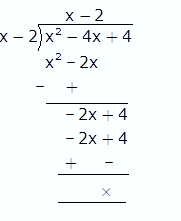Here quotient is x-2 and remainder is 0

4. Given (x2-4) by (x+2)

On dividing polynomial by a binomial we have divide every variables of polynomial

By binomial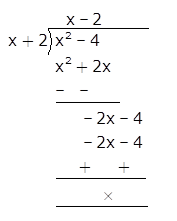Here quotient is x-2 and remainder is 0

5. Given (x2+12x+35) by (x+7)

On dividing polynomial by a binomial we have divide every variables of polynomial

By binomial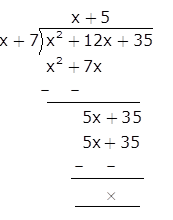Here quotient is x+5 and remainder is 0

6. Given (15x2+x-6) by (3x+2)

On dividing polynomial by a binomial we have divide every variables of polynomial

By binomial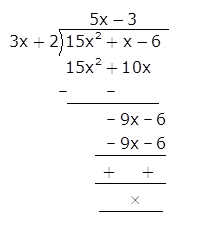Here quotient is 5x-3 and remainder is 0

7. Given (14x2-53x+45) by (7x-9)

On dividing polynomial by a binomial we have divide every variables of polynomial

By binomial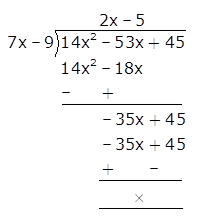Here quotient is 2x-5 and remainder is 0

## Exercise 6D

1. Find each of the following products:

(i) (x + 6) (x +6)

(ii) (4x +5y) (4x + 5y)

(iii) (7a +9b) (7a +9b)

(iv) ((2/3) x + (4/5) y) ((2/3) x + (4/5) y)

(v) (x2+7) (x2+7)

(vi) ((5/6) a2+2) ((5/6) a2+2)

Solution:

(i) Given that (x + 6) (x +6)

But we can write the given expression as (x + 6) (x +6) = (x + 6)2

But we have (a + b)2=a2+2ab+b2

On applying above identity in the given expression we get,

(x + 6)2= x2+2 x (6) + 62

(x + 6)2= x2+12 x + 36

(ii) Given that (4x + 5y) (4x +5y)

But we can write the given expression as (4x + 5y) (4x +5y) = (4x + 5y)2

But we have (a + b)2=a2+2ab+b2

On applying above identity in the given expression we get,

(4x + 5y)2= (4x)2+2 (4x) (5y) + (5y)2

(4x + 5y)2= 16x2+40 xy + 25y2

(iii) Given that (7a +9b) (7a +9b)

But we can write the given expression as (7a +9b) (7a +9b) = (7a +9b)2

But we have (a + b)2=a2+2ab+b2

On applying above identity in the given expression we get,

(7a +9b)2= (7a)2+2 (7a) (9b) + (9b)2

(7a +9b)2= 49a2+126 ab + 81b2

(iv) Given that ((2/3) x + (4/5) y) ((2/3) x + (4/5) y)

But we can write the given expression as

((2/3) x + (4/5) y) ((2/3) x + (4/5) y)= ((2/3) x + (4/5) y)2

But we have (a + b)2=a2+2ab+b2

On applying above identity in the given expression we get,

((2/3) x + (4/5) y)2= ((2/3) x)2+2 ((2/3)x) ((4/5)y) + ((4/5)y)2

((2/3) x + (4/5) y)2= (4/9) x2 + (16/15) xy + (16/25) y2

(v) Given that (x2+7) (x2+7)

But we can write the given expression as

(x2+7) (x2+7)= (x2+7)2

But we have (a + b)2=a2+2ab+b2

On applying above identity in the given expression we get,

(x2+7)2= (x2)2+2 ((x2) (7) + (7)2

(x2+7)2= x4+14x2 + 49

(vi) Given that ((5/6) a2+2) ((5/6) a2+2)

But we can write the given expression as

((5/6) a2+2) ((5/6) a2+2)= ((5/6) a2+2)2

But we have (a + b)2=a2+2ab+b2

On applying above identity in the given expression we get,

((5/6) a2+2)2= ((5/6) a2 )2+2 ((5/6)a2 ) (2) + (2)2

((5/6) a2+2)2= (25/36) a4+ (10/3) a2+ 4

2. Find each of the following products:

(i) (x – 4) (x – 4)

(ii) (2x-3y) (2x-3y)

(iii) ((3/4) x – (5/6) y) ((3/4) x + (5/6) y)

(iv) (x – (3/x) ) ( x – (3/x) )

(v) ((1/3) x2 – 9) ((1/3) x2 – 9)

(vi) ((1/2) y2 – (1/3) y) ((1/2) y2 – (1/3)y)

Solution:

(i) Given (x – 4) (x – 4)

But we can write the given expression as (x – 4) (x -4) = (x – 4)2

But we have (a – b)2=a2-2ab+b2

On applying above identity in the given expression we get,

(x – 4)2= x2-2 x (4) + 42

(x – 4)2= x2-8 x + 16

(ii) Given (2x-3y) (2x-3y)

But we can write the given expression as (2x – 3y) (2x -3y) = (2x – 3y)2

But we have (a – b)2=a2-2ab+b2

On applying above identity in the given expression we get,

(2x – 3y)2= 4x2-2 (2x) (3y) + 9y2

(2x – 3y)2= 4x2-12 xy + 9y2

(iii) Given that ((3/4) x – (5/6) y) ((3/4) x + (5/6) y)

But we can write the given expression as

((3/4) x – (5/6) y) ((3/4) x + (5/6) y)= ((3/4) x – (5/6) y)2

But we have (a – b)2=a2-2ab+b2

On applying above identity in the given expression we get,

((3/4) x – (5/6) y)2= ((3/4) x)2-2 ((3/4) x) ((5/6) y) + ((5/6) y)2

((3/4) x – (5/6) y)2= (9/16) x2– (15/12) xy + (25/36)y2

(iv) Given that (x – (3/x)) (x – (3/x))

But we can write the given expression as

(x – (3/x)) (x – (3/x))= (x – (3/x))2

But we have (a – b)2=a2-2ab+b2

On applying above identity in the given expression we get,

(x – (3/x))2= (x)2-2 (x) (3/x) + (3/x)2

(x – (3/x))2= x2– 6+ (9/x2)

(v) Given that ((1/3) x2 – 9) ((1/3) x2 – 9)

But we can write the given expression as

((1/3) x2 – 9) ((1/3) x2 – 9)= ((1/3) x2 – 9)) 2

But we have (a – b)2=a2-2ab+b2

On applying above identity in the given expression we get,

((1/3) x2 – 9))2= ((1/3) x)2-2 ((1/3) x2 ) (9) + (9)2

((1/3) x2 – 9))2= ((1/9) x4)- 6x2 +81

(vi) Given that ((1/2) y2 – (1/3) y) ((1/2) y2 – (1/3) y)

But we can write the given expression as

((1/2) y2 – (1/3) y) ((1/2) y2 – (1/3) y) = ((1/2) y2 – (1/3) y) 2

But we have (a – b)2=a2-2ab+b2

On applying above identity in the given expression we get,

((1/2) y2 – (1/3) y) 2= ((1/2) y2)2 -2 ((1/2) y2) (1/3) + (1/3) y2

((1/2) y2 – (1/3) y) 2= ((1/4) y4– y3 (1/3) + (1/9) y2

3. Expand:

(i) (8a+3b)2

(ii) (7x+2y)2

(iii) (5x+11)2

(iv) ((a/2) + (2/a))2

(v) ((3x/4) + (2y/9))2

(vi) (9x-10)2

(vii) (x2y – yz2)-2

(viii) ((x/y)-((y/x))2

Solution:

(i) Given (8a+3b)2

According to the identity (a + b)2=a2+2ab+b2 we have to expand the given expression,

(8a+3b)2= (8a)2+2 (8a)(3b)+(3b)2

(8a+3b)2= 64a2+48ab+9b2

(ii) Given (7x+2y)2

According to the identity (a + b)2=a2+2ab+b2 we have to expand the given expression,

(7x+2y)2= (7x)2+2 (7x)(2y)+(2y)2

(7x+2y)2= 49x2+28xy+4y2

(iii) Given (5x+11)2

According to the identity (a + b)2=a2+2ab+b2 we have to expand the given expression,

(5x+11)2= (5x)2+2 (5x)(11)+(11)2

(5x+11)2= 25x2+110x+121

(iv) Given ((a/2) + (2/a))2

According to the identity (a + b)2=a2+2ab+b2 we have to expand the given expression,

((a/2) + (2/a))2= (a/2)2+2 (a/2) (2/a)+ (2/a)2

((a/2) + (2/a))2= a2/4+2+4/a2

(v) Given ((3x/4) + (2y/9))2

According to the identity (a + b)2=a2+2ab+b2 we have to expand the given expression,

((3x/4) + (2y/9))2= (3x/4)2+2 (3x/4) (2y/9)+ (2y/9)2

((3x/4) + (2y/9))2= 9x2/16+ (1/3) xy + (4y 2/81)

(vi) Given (9x-10)2

According to the identity (a – b)2=a2-2ab+b2 we have to expand the given expression,

(9x-10)2= (9x)2-2 (9x)(10)+(10)2

(9x-10)2= 81x2-180x+100

(vii) Given (x2y – yz2)-2

According to the identity (a – b)2=a2-2ab+b2 we have to expand the given expression,

(x2y – yz2)-2= (x2y)2-2 (x2y)( yz2)+( yz2)2

(x2y – yz2)-2= x4y2-2x2y2z2+y2z4

(viii) Given ((x/y)-((y/x))2

According to the identity (a – b)2=a2-2ab+b2 we have to expand the given expression,

((x/y)-((y/x))2= (x/y)2-2 (x/y)( y/x)+(y/x)2

((x/y)-((y/x))2= x2/y2-2+y2/x2

4. Find each of the following products:

(i) (x+3) (x-3)

(ii) (2x+5) (2x-5)

(iii) (8+x) (8-x)

(iv) (7x+11y) (7x-11y)

(v) (5x2 + (3/4)y2) (5x2-(3/4)y2)

(vi) ((4x/5)-(5y/3)) ((4x/5) + (5y/3))

(vii) ((x + (1/x)) ((x-(1/x))

(viii) ((1/x) + (1/y)) ((1/x)-(1/y))

(ix) (2a + (3/b)) (2a – (3/b))

Solution:

(i) Given (x+3) (x-3)

By using the formula (a + b) (a – b) = a2 – b2

Applying the formula we get

(x+3) (x-3) =x2-32

(x+3) (x-3) = x2-9

(ii) Given (2x+5) (2x-5)

By using the formula (a + b) (a – b) = a2 – b2

Applying the formula we get

(2x+5) (2x-5) = (2x)2-52

(2x+5) (2x-5)= 4x2-25

(iii) Given (8+x) (8-x)

By using the formula (a + b) (a – b) = a2 – b2

Applying the formula we get

(8+x) (8-x) = (8)2-x2

(8+x) (8-x)= 64 – x2

(iv) Given (7x+11y) (7x-11y)

By using the formula (a + b) (a – b) = a2 – b2

Applying the formula we get

(7x+11y) (7x-11y) = (7x)2-(11y)2

(7x+11y) (7x-11y)= 49x2-121y2

(v) Given (5x2 + (3/4)y2) (5x2-(3/4)y2)

By using the formula (a + b) (a – b) = a2 – b2

Applying the formula we get

(5x2 + (3/4) y2) (5x2-(3/4) y2)= (5x2)2-((3/4) y2)2

(5x2 + (3/4) y2) (5x2-(3/4) y2)= 25x4-(9/16) y4

(vi) Given ((4x/5)-(5y/3)) ((4x/5) + (5y/3))

By using the formula (a + b) (a – b) = a2 – b2

Applying the formula we get

((4x/5)-(5y/3)) ((4x/5) + (5y/3))= (4x/5)2-((5y/3)2

((4x/5)-(5y/3)) ((4x/5) + (5y/3))= (16x2/25)-(25y2/15)

(vii) Given ((x + (1/x)) ((x-(1/x))

By using the formula (a + b) (a – b) = a2 – b2

Applying the formula we get

((x + (1/x)) ((x-(1/x)) = (x)2-(1/x)2

((x + (1/x)) ((x-(1/x)) = (x2)-(1/x2)

(viii) Given ((1/x) + (1/y)) ((1/x)-(1/y))

By using the formula (a + b) (a – b) = a2 – b2

Applying the formula we get

((1/x) + (1/y)) ((1/x)-(1/y))= (1/x)2-(1/y)2

((1/x) + (1/y)) ((1/x)-(1/y))= (1/x2)-(1/y2)

(ix) Given (2a + (3/b)) (2a – (3/b))

By using the formula (a + b) (a – b) = a2 – b2

Applying the formula we get

(2a + (3/b)) (2a – (3/b))= (2a)2-(3/b)2

(2a + (3/b)) (2a – (3/b))= 4a2-9/b2

5. Using the formula for squaring a binomial, evaluate the following:

(i) (54)2

(ii) (82)2

(iii) (103)2

(iv) (704)2

Solution:

(i) Given (54)2

But we can write 54 as 50+4

And also we know that (a + b)2 = a2+2ab+b2

By applying the above identity we get

(54)2 = (50+4)2= 502+2(50) (4) +42

(50+4)2=2500+400+16=2916

(ii) Given (82)2

But we can write 82 as 80+2

And also we know that (a + b)2 = a2+2ab+b2

By applying the above identity we get

(82)2 = (80+2)2= 802+2(80) (2) +22

(80+2)2=6400+320+4=6724

(iii) Given (103)2

But we can write 103 as 100+3

And also we know that (a + b)2 = a2+2ab+b2

By applying the above identity we get

(103)2 = (100+3)2= 1002+2(100) (3) +32

(100+3)2=10000+600+9=10609

(iv) Given (704)2

But we can write 704 as 700+4

And also we know that (a + b)2 = a2+2ab+b2

By applying the above identity we get

(704)2 = (700+4)2= 7002+2(700) (4) +42

(700+4)2=490000+2800+16=495616

6. using the formula for squaring a binomial, evaluate the following:

(i) (69)2

(ii) (78)2

(iii) (197)2

(iv) (999)2

Solution:

(i) Given (69)2

But we can write 69 as 70-1

And also we know that (a – b)2 = a2-2ab+b2

By applying the above identity we get

(69)2 = (70-1)2= 702-2(70) (1) +12

(70-1)2=4900-140+1=4761

(ii) Given (78)2

But we can write 78 as 80-2

And also we know that (a – b)2 = a2-2ab+b2

By applying the above identity we get

(78)2 = (80-2)2= 802-2(80) (2) +22

(80-2)2=6400-320+4=6084

(iii) Given (197)2

But we can write 197 as 200-3

And also we know that (a – b)2 = a2-2ab+b2

By applying the above identity we get

(197)2 = (200-3)2= 2002-2(200) (3) +32

(200-3)2=40000-1200+9=38809

(iv) Given (999)2

But we can write 999 as 1000-1

And also we know that (a – b)2 = a2-2ab+b2

By applying the above identity we get

(999)2 = (1000-1)2= 10002-2(1000) (1) +12

(1000-1)2=1000000-2000+1=998001

## Exercise 6E

Select the correct answer in each of the following:

1. The sum of (6a+4b-c+3), (2b-3c+4), (11b-7a+2c-1) and (2c-5a-6) is

(a) (4a-6b+2) (b) (-3a+14b-3c+2) (c) (-6a+17b) (d) (-6a+6b+c-4)

Solution:

(c) (-6a+17b)

Explanation:

Given (6a+4b-c+3), (2b-3c+4), (11b-7a+2c-1) and (2c-5a-6)

To add the given expression we have arrange them column wise is given below: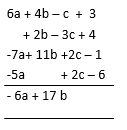2. (3q+7p2-2r3+4) – (4p2-2q+7r3-3) =?

(a)(p2+2q+5r3+1) (b) (11p2+q+5r3+1) (c) (-3p2-5q+9r3-7) (d) (3p2+5q-9r3+7)

Solution:

(d) (3p2+5q-9r3+7)

Explanation:

Given (3q+7p2-2r3+4) – (4p2-2q+7r3-3)

According to the rules of subtraction of algebraic equations, we have negative sign will

Becomes positive and so we have to keep the big numerical sign.

Now arrange the variables in rows we get

(3q+7p2-2r3+4) – (4p2-2q+7r3-3) = (3p2+5q-9r3+7)

3. (x+5) (x-3) =?

(a) x2+5x-15 (b) x2-3x-15 (c) x2+2x+15 (d) x2+2x-15

Solution:

(d) x2+2x-15

Explanation:

Given (x+5) (x-3)

By solving in horizontal method we get

(x+5) (x-3)=x (x-3) + 5 (x-3)

(x+5) (x-3)= x2-3x+5x-15

(x+5) (x-3)= x2+2x-15

4. (2x+3) (3x-1) =?

(a) (6x2+8x-3) (b) (6x2+7x-3) (c) (6x2-7x-3) (d) (6x2-7x+3)

Solution:

(b) (6x2+7x-3)

Explanation:

Given (2x+3) (3x-1)

By solving in horizontal method we get

(2x+3) (3x-1)= 2x (3x-1) + 3 (3x-1)

(2x+3) (3x-1)= (6x2+7x-3)

5. (x+4) (x+4) =?

(a) (x2+16) (b) (x2+4x+16) (c) (x2+8x+16) (d) (x2+16x)

Solution:

(c) (x2+8x+16)

Explanation:

Given (x+4) (x+4)=(x+4)2

By expanding the given expression by using (a + b)2 = a2+2ab+b2 we get

(x+4)2 = x2+2(x) (4)+42 = x2+8x+16

6. (x-6) (x-6) =?

(a) (x2-36) (b) (x2+36) (c) (x2-6x+36) (d) (x2-12x+36)

Solution:

(d) (x2-12x+36)

Explanation:

Given (x-6) (x-6)=(x-6)2

By expanding the given expression by using (a – b)2 = a2-2ab+b2 we get

(x-6)2 = x2-2(x) (6)+62 = x2-12x+36

## RS Aggarwal Solutions for Class 8 Maths Chapter 6 – Operations on Algebraic Expressions

Chapter 6, Operations on Algebraic Expressions, contains 5 exercises. RS Aggarwal Solutions given here contains the answers to all the questions present in these exercises. Let us have a look at some of the concepts that are being discussed in this chapter.

• Subtraction of Algebraic Expressions
• Multiplication of Algebraic Expressions
• Division of Algebraic Expressions
• Special Identities
• Applications of Identities

Exercise 6A

Exercise 6B

Exercise 6C

Exercise 6D

Exercise 6E

### Chapter Brief RS Aggarwal Solutions for Class 8 Maths Chapter 6 – Operations on Algebraic Expressions

The RS Aggarwal Solutions for Class 8 Maths Chapter 6 – Operations on Algebraic Expressions deals with basic operations such as addition, subtraction, multiplication and division on algebraic expressions. It also deals with some special identities which help in solving problems directly and quickly. These are basic identities which we are using in higher studies.